# US7907512B1 - OFDM and SC-OFDM QLM - Google Patents

## Info

Publication number
US7907512B1
US7907512B1 US12/587,687 US58768709A US7907512B1 US 7907512 B1 US7907512 B1 US 7907512B1 US 58768709 A US58768709 A US 58768709A US 7907512 B1 US7907512 B1 US 7907512B1
Authority
US
United States
Prior art keywords
data
qlm
communications
ofdm
subband
Prior art date
Legal status (The legal status is an assumption and is not a legal conclusion. Google has not performed a legal analysis and makes no representation as to the accuracy of the status listed.)
Active
Application number
US12/587,687
Inventor
Urbain Alfred von der Embse
Original Assignee
Urbain A. von der Embse
Priority date (The priority date is an assumption and is not a legal conclusion. Google has not performed a legal analysis and makes no representation as to the accuracy of the date listed.)
Filing date
Publication date
Priority to US12/380,668 priority Critical patent/US7855995B1/en
Application filed by Urbain A. von der Embse filed Critical Urbain A. von der Embse
Priority to US12/587,687 priority patent/US7907512B1/en
Application granted granted Critical
Publication of US7907512B1 publication Critical patent/US7907512B1/en
Active legal-status Critical Current
Anticipated expiration legal-status Critical

• 239000010410 layers Substances 0.000 claims abstract description 97
• 238000007476 Maximum Likelihood Methods 0.000 claims abstract description 43
• 230000000051 modifying Effects 0.000 claims abstract description 40
• 230000002596 correlated Effects 0.000 claims description 24
• 239000011159 matrix materials Substances 0.000 claims description 14
• 230000001702 transmitter Effects 0.000 claims description 14
• 230000000875 corresponding Effects 0.000 claims description 12
• 239000000562 conjugates Substances 0.000 claims description 9
• 239000000969 carrier Substances 0.000 claims description 6
• 230000001360 synchronised Effects 0.000 claims description 4
• 239000002356 single layers Substances 0.000 claims description 3
• 230000003595 spectral Effects 0.000 claims description 3
• 238000005192 partition Methods 0.000 claims description 2
• 238000005516 engineering processes Methods 0.000 description 5
• 238000000926 separation method Methods 0.000 description 4
• 238000010276 construction Methods 0.000 description 2
• 238000009795 derivation Methods 0.000 description 2
• 238000000034 methods Methods 0.000 description 2
• 238000004364 calculation methods Methods 0.000 description 1
• 238000005070 sampling Methods 0.000 description 1

## Images

••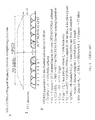•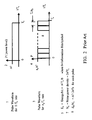•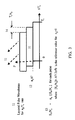•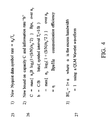•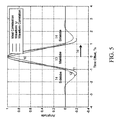•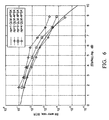•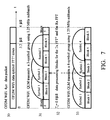•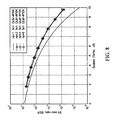•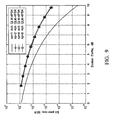•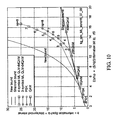•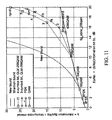•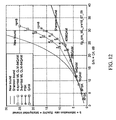•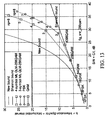•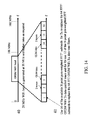•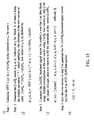•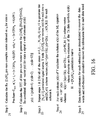•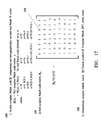•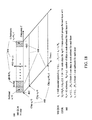••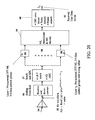## Classifications

• HELECTRICITY
• H04ELECTRIC COMMUNICATION TECHNIQUE
• H04LTRANSMISSION OF DIGITAL INFORMATION, e.g. TELEGRAPHIC COMMUNICATION
• H04L5/00Arrangements affording multiple use of the transmission path
• H04L5/0001Arrangements for dividing the transmission path
• H04L5/0014Three-dimensional division
• HELECTRICITY
• H04ELECTRIC COMMUNICATION TECHNIQUE
• H04JMULTIPLEX COMMUNICATION
• H04J13/00Code division multiplex systems
• H04J13/0007Code type
• H04J13/004Orthogonal
• H04J13/0048Walsh
• HELECTRICITY
• H04ELECTRIC COMMUNICATION TECHNIQUE
• H04JMULTIPLEX COMMUNICATION
• H04J13/00Code division multiplex systems
• H04J13/10Code generation
• H04J13/12Generation of orthogonal codes
• HELECTRICITY
• H04ELECTRIC COMMUNICATION TECHNIQUE
• H04LTRANSMISSION OF DIGITAL INFORMATION, e.g. TELEGRAPHIC COMMUNICATION
• H04L1/00Arrangements for detecting or preventing errors in the information received
• H04L1/004Arrangements for detecting or preventing errors in the information received by using forward error control
• H04L1/0045Arrangements at the receiver end
• HELECTRICITY
• H04ELECTRIC COMMUNICATION TECHNIQUE
• H04LTRANSMISSION OF DIGITAL INFORMATION, e.g. TELEGRAPHIC COMMUNICATION
• H04L25/00Baseband systems
• H04L25/02Details ; Arrangements for supplying electrical power along data transmission lines
• H04L25/03Shaping networks in transmitter or receiver, e.g. adaptive shaping networks ; Receiver end arrangements for processing baseband signals
• H04L25/03006Arrangements for removing intersymbol interference
• H04L25/03178Arrangements involving sequence estimation techniques
• H04L25/03203Trellis search techniques
• HELECTRICITY
• H04ELECTRIC COMMUNICATION TECHNIQUE
• H04LTRANSMISSION OF DIGITAL INFORMATION, e.g. TELEGRAPHIC COMMUNICATION
• H04L27/00Modulated-carrier systems
• H04L27/26Systems using multi-frequency codes
• H04L27/2601Multicarrier modulation systems
• H04L27/2626Arrangements specific to the transmitter
• H04L27/2627Modulators
• HELECTRICITY
• H04ELECTRIC COMMUNICATION TECHNIQUE
• H04LTRANSMISSION OF DIGITAL INFORMATION, e.g. TELEGRAPHIC COMMUNICATION
• H04L27/00Modulated-carrier systems
• H04L27/26Systems using multi-frequency codes
• H04L27/2601Multicarrier modulation systems
• H04L27/2647Arrangements specific to the receiver
• H04L27/2649Demodulators
• HELECTRICITY
• H04ELECTRIC COMMUNICATION TECHNIQUE
• H04LTRANSMISSION OF DIGITAL INFORMATION, e.g. TELEGRAPHIC COMMUNICATION
• H04L5/00Arrangements affording multiple use of the transmission path
• H04L5/02Channels characterised by the type of signal
• H04L5/04Channels characterised by the type of signal the signals being represented by different amplitudes or polarities, e.g. quadriplex

## Abstract

This invention provides a method for increasing the data rates supported by WiFi, WiMax, LTE communications using OFDM and SC-OFDM data symbol waveforms, by using quadrature layered modulation QLM which layers communications channels with a differentiating parameter for each layer that enables a demodulation algorithm to recover the data symbols in each layer, and supports higher data symbol rates then allowed by the Nyquist rate. A maximum likelihood (ML) QLM demodulation algorithm supports data rates to 4.75×57=271 Mbps compared to the current OFDM WiFi standard 57 Mbps with similar increases for WiMax, LTE. Multi-scale (MS) coding can be implemented to spread each data symbol over the OFDM band and over the 4 μs data packet to optimize BER performance. Computationally efficient signal processing for transmit and receive for OFDM and SC-OFDM are disclosed and Matlab direct error count Monte Carlo bit error rate simulations are evaluated to predict performance.

## Description

This patent filing is a continuation in part of application Ser. No. 12/380,668 filed on Mar. 3, 2009.

BACKGROUND OF THE INVENTION

I. Field of the Invention

The present invention relates to cellular communications and also relates to the Nyquist rate for data symbol transmission, the Shannon bound on communications capacity, and symbol modulation and demodulation for high-data-rate satellite, airborne, wired, wireless, and optical communications and includes all of the communications symbol modulations and the future modulations for single links and multiple access links which include electrical and optical wired, mobile, point-to-point, point-to-multipoint, multipoint-to-multipoint, cellular, multiple-input multiple-output MIMO, and satellite communication networks. In particular it relates to WiFi, WiMax and long-term evolution LTE for cellular communications and satellite communications. WiFi, WiMax use orthogonal frequency division multiplexing OFDM on both links and LTE uses SC-OFDM on the uplink from user to base station and OFDM on the downlink form base station to user. WiMax occupies a larger frequency band than WiFi and both use OFDM waveforms. SC-OFDM is a single carrier orthogonal waveform version of OFDM which uses orthogonal frequency subbands of varying widths.

II. Description of the Related Art

Two fundamental bounds on communications are the Nyquist rate and the Shannon capacity theorem. The Nyquist rate is the complex digital sampling rate equal to B that is sufficient to include all of the information within a frequency band B. For communications, equivalent expressions for the Nyquist rate bound are defined in equations (1).
T s≧1/B  (1)
BTS≧1
wherein 1/Ts is the data symbol transmission rate in the frequency band B which means Ts is the spacing between the data symbols.

The Shannon bound for the maximum data rate C is a bound on the corresponding number of information bits per symbol b as well as a bound on the communications efficiency η and is complemented by the Shannon coding theorem, and are defined in equations (2).

3 Shannon coding theorem for the information bit rate Rb

• For Rb<C there exists codes which support reliable communications
• For Rb>C there are no codes which support reliable communications
wherein Eb/No is the ratio of energy per information bit Eb to the noise power density No, max{b} is the maximum value of the number of information bits per symbol b and also is the information rate in Bps/Hz, and since the communications efficiency η=b/(TSB) in bits/sec/Hz it follows that maximum values of b and η are equal. Derivation of the equation for Eb/No uses the definition Eb/No=(S/N)/b in addition to 1 and 2. Reliable communications in the statement of the Shannon coding theorem 3 means an arbitrarily low bit error rate BER.

OFDM is defined in FIG. 1 for the WiFi 802.16 standard power spectrum in 1,2 which implements the inverse FFT (IFFT=FFT−1) to generate OFDM (or equivalently OFDMA which is orthogonal frequency division multiple access to emphasize the multiple access applications) data symbol tones 2 over the first 3.2 μs of the 4 μS data packet in 30 in FIG. 7 with some rolloff of the tones at their ends for spectral containment. Data symbol tones are modulated with 4 PSK, 16QAM, 64QAM, 256QAM depending on the transmission range and data rate and for 256QAM using the code rate option R=¾ yields the information rate b=6 Bps/Hz for the WiFi standard, with other code options available. The N=64 point FFT−1 generates N=64 tones in 2 over the 20 MHz WiFi band with 48 tones used for data transmission. In 3 the WiFi parameters are defined including a calculation of the maximum data rate Rb=57 Mbps. Later versions of WiFi allow WiFi bands of 1.25, 5, 10, 20 MHz corresponding to N=4, 16, 32, 64. For this representative OFDM WiFi QLM disclosure we are considering the WiFi standard in FIG. 1. The maximum data rate supported by WiFi standard is calculated in 3 to be ˜57 Mbps using 256QAM modulation and wherein “˜” represents an approximate value. OFDM uses pulse waveforms in time and relies on the OFDM tone modulation to provide orthogonality. SC-OFDM is a pulse-shaped OFDM that uses shaped waveforms in time to roll-off the spectrum of the waveform between adjacent channels to provide orthogonality, allows the user to occupy subbands of differing widths, and uses a different tone spacing, data packet length, and sub-frame length compared to OFDM for WiFi, WiMax.

SUMMARY OF THE INVENTION

This invention introduces a maximum likelihood ML demodulation architecture and implementation of a quadrature layered modulation QLM for OFDM and SC-OFDM modulations to provide a method for increasing the data rates. QLM for OFDM WiFi provides a method for increasing the data rates to 4.75× WiFi maximum data rate with current technology (4.75 times the WiFi maximum rate) and to 6× WiFi maximum data rate with technology advances. QLM provides similar increases in data rate for OFDM WiMax and SC-OFDM LTE. QLM supports data symbol rates that can be multiples of the Nyquist rate and communications data rates that can be multiples of the Shannon bound.

A representative OFDM QLM architecture using ML demodulation is disclosed in this invention for WiFi standard and the transmit and receive signal processing algorithms and supporting block diagrams are developed to illustrate the architecture and implementation. This architecture is directly applicable to WiMax by increasing the number of OFDM tones to occupy the increased WiMax frequency band and also is directly applicable to SC-OFDM since the OFDM QLM ML architecture uses a pulse-shaped OFDM which partitions the frequency band into orthogonal subbands that can be combined to enable users to use differing frequency bands to implement SC-OFDM.

QLM is a layered topology for transmitting higher data rates than possible with a single layer of communications and is implemented by transmitting each layer with a differentiating parameter which enables separation and decoding of each layer. For a representative OFDM architecture the OFDM WiFi QLM transmits the QLM signals over a set of subbands which together occupy the same frequency band as the WiFi standard 48 data symbol modulated FFT−1 tones and over the WiFi 4 μs data packet. Computationally efficient fast multi-channel FFT−1 and FFT algorithms generate the subband QLM data symbols for transmission and implement the receive detection followed by maximum likelihood ML demodulation, which can be implemented with a chip architecture that supports both OFDM WiFi and the OFDM WiFi QLM in this invention disclosure as well as both OFDM WiMax and OFDM WiMax QLM with an increase in the frequency band, and with parameter changes the chip architecture supports both SC-OFDM LTE and SC-OFDM LTE QLM uplinks and both OFDMA LTE and OFDMA LTE QLM downlinks. Monte Carlo Matlab direct error count bit-error-rate BER simulations for ML demodulation used in OFDM WiFi QLM demonstrate the QLM performance.

A multi-scale MS code can be implemented with modest complexity in order to improve the bit-error-rate BER performance of OFDM WiFi QLM by spreading each transmitted data symbol over the OFDM WiFi QLM data band and over the 4 μs data packet. Jensen's inequality from mathematical statistics proves that this uniform spreading of the Tx signals using MS provides the best communications BER performance.

BRIEF DESCRIPTION OF THE SEVERAL VIEWS OF THE DRAWINGS

The above-mentioned and other features, objects, design algorithms, and performance advantages of the present invention will become more apparent from the detailed description set forth below when taken in conjunction with the drawings and performance data wherein like reference characters and numerals denote like elements and in which:

FIG. 1 describes the OFDM waveform for the WIFi standard.

FIG. 2 describes how to increase the data rate using a pulse waveform.

FIG. 3 describes how QLM increases the data rate for a pulse waveform at a constant frequency bandwidth.

FIG. 4 presents the equations for the communications capacity predicted by the new bound, the new Nyquist data symbol rate, and the BTS product for a Wavelet waveform.

FIG. 5 calculates the ideal pulse correlation, candidate waveform Ψ time response, and the Ψ correlation.

FIG. 6 presents the measured bit error rate BER performance of a pulse waveform with 4 PSK modulation for QLM layers np=1,2,3,4 using a Trellis demodulation algorithm.

FIG. 7 describes the OFDM WiFi QLM 3-data symbol group and 4-data symbol group in each of the subbands and over the 4 μs WiFi data packet.

FIG. 8 presents the measured BER performance of a pulse waveform with 4 PSK modulation for QLM layers np=1,2,4,8 for a 3-data symbol group using a maximum likelihood ML demodulation algorithm.

FIG. 9 presents the measured BER performance of a pulse waveform with 4 PSK modulation for QLM layers np=1,2,4,8 for a 4-data symbol group using a ML demodulation algorithm.

FIG. 10 for the OFDM architecture calculates the information bits b per symbol interval equivalently expressed as Bps/Hz versus Eb/No for the new bound, Shannon bound, for PSK, QAM with turbo coding to bring the performance to essentially equal to the Shannon bound, and for QLM 3,4-data symbol group performance using 64QAM.

FIG. 11 for the OFDM architecture calculates the information bits b per symbol interval expressed as Bps/Hz versus Eb/No for the new bound, Shannon bound, for PSK, QAM with turbo coding to bring the performance to essentially equal to the Shannon bound, and for QLM 3,4-data symbol group performance using 256QAM.

FIG. 12 for the OFDM architecture calculates the information bits b per symbol expressed as Bps/Hz versus S/N=C/I for the new bound, Shannon bound, for PSK, QAM with turbo coding to bring the performance to essentially equal to the Shannon bound, and for QLM 3,4-data symbol group performance using 64QAM.

FIG. 13 for the OFDM architecture calculates the information bits b per symbol interval expressed as Bps/Hz versus S/N=C/I for the new bound, Shannon bound, for PSK, QAM with turbo coding to bring the performance to essentially equal to the Shannon bound, and for QLM 3,4-data symbol group performance using 256QAM.

FIG. 14 describes the partitioning of the OFDM WiFi standard 20 MHz band into 16 OFDM WiFi QLM subbands.

FIG. 15 presents the implementation steps in the transmitter to generate the OFDM WiFi QLM baseband signal vector starting with the input data symbols for the 4 μs data packet

FIG. 16 presents the implementation steps in the receiver to recover the estimated OFDM WiFi QLM input data symbols starting with the received OFDM WiFi QLM baseband signal vector for the 4 μs data packet.

FIG. 17 defines the complex Walsh codes and the generalized complex Walsh codes.

FIG. 18 describes the multi-scale MS encoding of each layer of OFDM WiFi QLM which spreads each data symbol vector within the subbands and over the subbands.

FIG. 19 is a representative transmitter implementation block diagram for the OFDM WiFi QLM mode.

FIG. 20 is a representative receiver implementation block diagram for the OFDM WiFi QLM mode.

DETAILED DESCRIPTION OF THE INVENTION

OFDM and SC-OFDM applications of quadrature layered modulation QLM in this invention disclosure are illustrated by the WiFi 802.16 standard which uses OFDM on both uplinks and downlinks between the user and base station for cellular communications as well as for communications with satellites. OFDM WiFi QLM replaces the OFDM orthogonal data symbol tones with orthogonal subbands which are the same architecture as SC-OFDM used for the LTE uplink. This means the OFDM WiFi QLM architecture is directly applicable to WiMax by simply increasing the number of subbands since they both use the same OFDM and 4 μs data packets with WiMax using a larger frequency band, and also is directly applicable to the LTE uplink since the OFDM WiFi QLM orthogonal subbands partition the frequency spectrum using the same architecture as SC-OFDM for LTE to allow the various users to be assigned differing orthogonal frequency subbands across the frequency band and the QLM data symbol waveforms in these subbands are SC-OFDM subband shaped waveforms used for LTE. The OFDM WiFi QLM architecture is directly applicable to LTE downlinks which use OFDM.

OFDM WiFi QLM uses maximum likelihood ML demodulation of the quadrature layered modulation QLM received correlated data symbols to support an architecture and implementation for QLM communications using the WiFi 4 μs data packet over the 20 MHz WiFi band for the WiFi standard and with obvious extensions to the other WiFi versions.

FIG. 2 introduces QLM by considering an ideal pulse waveform in the time domain. In 1 the pulse waveform is transmitted at the data symbol rate equal to 1/Ts=B where Ts is the pulse length, B is the bandwidth, the signal power level 2 is P=A2 where “A” is the signal amplitude, and the pulse modulation is phase shift keying PSK with “b” information bits per data symbol. To increase the data symbol rate to np/Ts and the information rate to npb/Ts, the pulse waveform is shortened 3 to Ts/np which increases the bandwidth to npB wherein B=1/Ts and requires the transmitted power to be increased 4 to P=npA2 in order to keep the same pulse energy per bit 5 is Eb=A2Ts/b. The corresponding energy-per-bit to noise power ratio 6 is Eb/No A2/2σ2b where 7 No=2σ2Ts is the noise power density and 2σ2 is the “mean square” level of communication noise.

FIG. 3 implements this increase in the data symbol rate using QLM communications without changing the bandwidth of the pulse waveform by extending the pulses 8 in FIG. 2 over the original pulse length Ts and layering these extended data symbol waveforms on top of each other 11 while occupying the same bandwidth B=1/Ts. The pulse waveforms in each layer 13 have Eb/No values equal to np times the original Eb/No=A2/2σ2b due to the stretching of each pulse over Ts without changing the power level of the pulse. The layers are time synchronized for transmission at ΔTs=Ts/np, 2ΔTs . . . , (np−1)ΔTs offsets 14 respectively for layers 2, 3, . . . , (np−1) relative to the 1st layer at zero offset. This means the signal-to-noise power S/N over B=1/Ts is equal to np^2 times the original S/N due to the addition of the np pulse power levels 12 over each Ts interval and the scaling of Eb/No by np. This scaling of Eb/No in each of the layered communications channels is summarized in equation (3) along with the corresponding scaling of the S/N over Ts. We find

$E b / N o = ⁢ n p ⁡ [ E b / N o ] ⁢ ⁢ for ⁢ ⁢ each ⁢ ⁢ layer ⁢ ⁢ or ⁢ ⁢ channel = ⁢ n p ⁡ [ A 2 / 2 ⁢ σ 2 ⁢ b ] S / N = ⁢ ∑ n p ⁢ E b / N o ⁢ ⁢ over ⁢ ⁢ n p ⁢ ⁢ layer ⁢ ⁢ or ⁢ ⁢ channels = ⁢ n p ⋀ 2 ⁡ [ S / N ] ( 3 )$
wherein [“o”] is the value of “o” for the communications channel when there is no layering.

FIG. 3 describes the layering of the communications channels for QLM and equation (3) defines the QLM scaling of the Eb/No and S/N. QLM is a layered topology for transmitting higher data rates than possible with each layer of communications and is implemented by transmitting each layer with a differentiating parameter which enables separation and decoding of each layer. Each layer or channel has a unique differentiating parameter such as time offset as in FIG. 3 and/or frequency offset. Each layer or channel obeys Shannon's laws when using QLM scaling in equations (3).

The equations for the non-optimized channel capacity in Bps and information bits b per symbol interval are the Shannon's bounds in 1,2 in equation (2) with the maximum “max” removed, with the [S/N] scaling in equations (3), and with the multiplication by “np” to account for the np layers. We find

$C = n p ⁢ B ⁢ ⁢ log 2 ⁡ [ 1 + ( S / N ) / n p ^ 2 ] Bps b = n p ⁢ ⁢ log 2 ⁡ [ 1 + ( S / N ) / n p ^ 2 ] Bps / Hz = Bits / symbol ⁢ ⁢ interval ( 4 )$
using the definition b=CB in Bps/Hz=Bits/symbol from 2 in equations (2) and observing that “Bits/symbol” in 2 is “Bits/symbol interval” for QLM and wherein it is understood that the C,b are non-optimized values with respect to the selection of the np.

New upper bounds on C, b, η and a new lower bound on Eb/No are derived in equations (5) by using equation (4) and equation (2). We find

$For ⁢ ⁢ R b < C ⁢ ⁢ there exists codes which support reliable communications For ⁢ ⁢ R b > C ⁢ ⁢ there are no codes which support reliable communications$ $⁢ max ⁢ { n p ⁢ / ⁢ T s } = ⁢ n p ⁢ B ⁢ ⁢ for ⁢ ⁢ n p ⁢ ⁢ layers ⁢ ⁢ of ⁢ ⁢ communications = ⁢ n p ⁢ x ⁢ ⁢ ( Nyquist ⁢ ⁢ rate ⁢ ⁢ for ⁢ ⁢ 1 ⁢ ⁢ channel )$
wherein the maximum values of C, max{b}, and max{η} of C, b, η are the respective maximums of the expressions in equation (4) with respect to np, the units of C, b, η are Bps, information bits/symbol interval, and Bps/Hz which means b is expressed in units Bps/Hz as well as in units of information bits/symbol interval, and the min{Eb/No} is the minimum of Eb/No with respect to np similar to the derivation in 2 in equations (2).

The new coding theorem in 4 in equations (5) states that C is the upper bound on the information data rate Rb in bits/second for which error correcting codes exist to provide reliable communications with an arbitrarily low bit error rate BER wherein C is defined in 1 in equations (5) and upgrades the Shannon coding theorem 3 in equations (1) using new capacity bound C in 1 in equations (5) and introduces the new data symbol rate 5 whose maximum value max{np/Ts} is np times the Nyquist rate for a single channel.

FIG. 4 restates the new communications bound in U.S. Pat. No. 7,391,819 in a format suitable for implementations. Listed are the new Nyquist rate 25, new bounds on C, b, η in 26, and the assumed bandwidth-time product in 27 with the note that the excess bandwidth a is zero α=0 for a QLM Wavelet waveform from U.S. Pat. No. 7,376,688 and filing Ser. No. 12/152,318.

QLM demodulation received signal processing synchronizes and removes the received waveform by performing a convolution of the received waveform encoded data symbol with the complex conjugate of this waveform, to detect the correlated data symbols. This convolution is a correlation of the waveform with itself as illustrated in FIG. 5 since the waveforms are real and symmetric. These correlated data signals are processed with a trellis algorithm to recover estimates of the encoded symbol data, or processed by a ML algorithm to recover estimates of the data symbols, or processed by a recursive relaxation algorithm or another demodulation algorithm to recover the transmitted data symbols.

FIG. 5 calculates the ideal triangular correlation 10, an example waveform 11 designated by Ψ, and the waveform Ψ correlation 12. Parameters of interest for this example square-root raised-cosine waveform are the waveform length L=3, M=16, and excess bandwidth α=0.22, the mainlobe 13 which extends over a 2Ts interval, and the sidelobes 14 which fall outside of the mainlobe. Parameter L is the waveform length in units of M=16 samples and M is the number of digital samples between adjacent waveforms at a Nyquist symbol rate=1/Ts for which Ts=MT where 1/T is the digital sample rate and α is a measure of the roll-off of the frequency response. The ideal triangular correlation is the correlation for the pulse waveform of length Ts in FIG. 3 and FIG. 5 demonstrates that for waveforms of interest for QLM the triangular correlation approximates the mainlobe correlations for QLM waveforms.

FIG. 6 measures the trellis decoding performance for uncoded 4-PSK for np=1 and for np=2,3,4 layers of QLM modulation by implementing the trellis symbol decoding algorithm in U.S. Pat. No. 7,391,819 and validates the QLM technology and scaling. Performance is plotted as bit error rate BER versus the normalized value (Eb/No)/np of the Eb/No for the new bound from equations (3),(5). Normalization means that for a given BER the (Eb/No)/np has the same value for all np. For example, this means that BER=0.001 requires (Eb/No)/np=6.8 dB and for np=1,2,4 this requires Eb/No=6.8+0=6.8, 6.8+3=9.8, 6.8+6=12.8 dB respectively. Measured performance values for np=2,3,4 are from a direct error count Monte Carlo simulation of the trellis algorithm and are plotted in FIG. 6 as discrete measurement points.

FIG. 7 is a representative OFDM WiFi QLM architecture and implementation used to illustrate the implementation and performance. The OFDM WiFi QLM architecture partitions the WIFi 20 MHz frequency band into 16 1.25 MHz subbands and uses the WiFi 4 μS data packet 30 to transmit the maximum likelihood ML 3,4-data symbol groups in each OFDM WiFi QLM data subband by partitioning the data packet into data processing blocks for implementation of the OFDM WiFi QLM for transmission Tx using a post-weighted inverse fast fourier transform N-point FFT−1 algorithm and for the receiver Rx using a pre-weighted fast fourier transform N-point FFT algorithm followed by a ML demodulation algorithm. These processing blocks 32 are the data symbol separation Ts=0.8 μs=NT for QLM transmission in the OFDM WiFi QLM subbands wherein 1/T is the sample rate (bandwidth) of the FFT−1 and FFT. Mainlobes of the representtive waveforms in FIG. 5 for the 3,4-data symbol groups are depicted in 31 and 33 and each extends over a 2Ts length as depicted in 13 in FIG. 5

In FIG. 7 the ns=3-data symbol group 31 and the ns=4-data symbol group 33 comprise 3 and 4 symbols labeled 1,2,3 and 1,2,3,4 respectively for the QLM ground layer np=1. The data symbols for the additional layers are overlayed as shown in FIG. 2 on data symbols 1,2 and 1,2,3 and extend over data symbol 3 and 4 respectively. This layering means that for np layers the reference positions for the data symbols are 1, 1+1/np, 1+2/np, . . . , 3 and 4 respectively corresponding to data symbols s=1, 2, . . . , Ns=(ns−1)np+1 wherein ns is the number of contiguous data symbols in the ground layer group, Ns is the number of data symbols in the ns-data symbol group for np layers, and the index s identifies the data symbols in the order they are processed in the transmitter and in the receiver.

FIG. 7 OFDM WiFi QLM architecture for the ns-data symbol groups allows the ML system equations to be written in equation (6) for each set of Ns detected correlated data symbols over the OFDM WiFi QLM 4 μs data packet in each of the subbands k and enables a ML demodulation algorithm to be implemented to recover the estimates of the data symbols. System equations (6) calculate the received Rx detected data symbol vector y(k) as a linear sum of the transmitted Tx data symbol vector x(k) multiplied by the correlation matrix H plus the Rx noise vector u(k). We find
y(k)=H×(k)+u(k)  (6)

wherein

• Y(k)=Ns×1 detected symbol vector. in subband k.
• H=Ns×Ns correlation matrix of data symbols
• x(k)=Ns×1 data symbol vector for layered channels in subband k
• u(k)=Ns×1 demodulation plus link noise vector for subband k
wherein the Rx data symbol vector y(k) elements are y(s|k) which is the Rx detected correlated signal for state s=symbol s for subband k, the data symbol vector x(k) elements are x(s|k) which is the Tx data symbol x(s|k) for symbol s, u(k) is the noise vector, and “x” is the multiply operator. In this disclosure the same notation will be used for the estimates x(k), x(s|k) and for the true values x(k),x(s|k) respectively with the interpretation defined in the text. Equation (7) lists the ML solution. We find

$1 ⁢ ⁢ ML ⁢ ⁢ Cost ⁢ ⁢ is : ⁢ J = ⁢ [ y ⁡ ( k ) - Hx ⁡ ( k ) ] ′ ⁢ Q - 1 ⁡ [ y ⁡ ( k ) - Hx ⁢ ( k ) ] = ⁢ ( - ) ⁢ exponent ⁢ ⁢ of ⁢ ⁢ the ⁢ ⁢ ML ⁢ ⁢ probability ⁢ ⁢ density ⁢ ⁢ function ⁢ 2 ⁢ ⁢ ML ⁢ ⁢ solution ⁢ ⁢ which ⁢ ⁢ minimizes ⁢ ⁢ J ⁢ ⁢ is : x ⁡ ( k ) = ⁢ [ H ′ ⁢ Q - 1 ⁢ H ] - 1 ⁢ H ′ ⁢ Q - 1 ⁢ y ⁡ ( k ) 3 ⁢ ⁢ The ⁢ ⁢ noise ⁢ ⁢ covariance ⁢ ⁢ is : Q = ⁢ E [ u ⁡ ( k ) ⁢ u ⁡ ( k ) ′ } 4 ⁢ ⁢ ML ⁢ ⁢ solution ⁢ ⁢ simplifies ⁢ ⁢ to : x ⁡ ( k ) = ⁢ H - 1 ⁢ y ⁡ ( k ) ( 7 )$
since the inverse H−1 exists for all applications of interest, and wherein H′ is the conjugate transpose of H, and 2σ2 is the mean-square data symbol detection noise.

FIGS. 8,9 measure the Monte Carlo bit error rate BER vs. the scaled (Eb/No)/np from equations (3) for the 3-data symbol and 4-data symbol groups in the OFDM WiFi QLM architecture in FIG. 7 with np=1,2,4,8 layers using 4 PSK symbol encoding and waveforms with ideal correlations in FIG. 5 and the measured data validates the QLM technology and scaling. These BER measurements also apply to Wavelet and other waveforms since their correlations closely approximate ideal correlations in FIG. 5. Measured BER performance losses compared to ideal 4 PSK are expected to be the same for all data symbol modulations including 8 PSK, 16QAM, 64QAM, 256QAM, 2048QAM since the ML demodulation estimates the data symbols in each layer independent of the data symbol modulation. The 4 PSK modulation was used as a convenient modulation to measure the ML demodulation loss which loss is expected to apply to all data symbol modulations.

FIGS. 10-13 calculate the OFDM WiFi QLM information rate b Bps/Hz (information bits per data symbol interval Ts) performance vs. Eb/No and S/N=C/I using the ML BER performance in FIGS. 8,9 measured for the ns=3,4-data symbol architectures in FIG. 7. FIG. 10 calculates the b Bps/Hz vs. Eb/No for ns=3,4-data symbol blocks using QLM-64QAM which reads “modulation 64QAM used for QLM”, FIG. 11 calculates the b Bps/Hz vs. Eb/No for ns=3,4-data symbol blocks using QLM-256QAM, FIG. 12 calculates the b Bps/Hz vs. S/N for ns=3,4-data symbol blocks using QLM-64QAM, and FIG. 13 calculates the b Bps/Hz vs. S/N for ns=3,4-data symbol blocks using QLM-256QAM which reads “modulation 256QAM used for QLM”. Supporting this performance data are the calculations for the Shannon bound in equations (2) and the new bound in equations (5), and the number of information bits per symbol b=bits=Bps/Hz versus measured Eb/No, S/N=C/I for 4 PSK, 8 PSK, 16QAM, 64QAM, 256QAM, 4096QAM. The 4 PSK, 8 PSK are 4-phase, 8-phase phase shift keying modulations which respectively encode 2,3 bits per symbol and 16QAM, 64QAM, 256QAM, 1024QAM are 16, 64, 256, 4096 state QAM modulations which respectively encode 4, 6, 8, 12 bits. For no coding the information bits per symbol b is equal to the modulation bits per symbol bs so that b=bs=2,3,4,6,8,12 bits per symbol=Bps/Hz respectively for 4 PSK, 8 PSK, 16QAM, 64QAM, 256QAM, 4096QAM. Assumed turbo coding performance provides a performance almost equal to the Shannon bound. The assumed coding rates R=3/4, 2/3, 3/4, 2/3, 3/4, 2/3 reduce the information bits per symbol to the respective values b=1.5,2,3,4,6,8 bits=Bps/Hz.

The OFDM WiFi QLM performance calculations in FIGS. 10-13 use the information rates 1, scaling laws 2, and demodulation losses 3 in equations (8). OFDM WiFi QLM data rates 1 are calculated in FIGS. 10-13 for the values of Eb/No and S/N calculated in 2 with demodulation losses in 3 subtracted from these calculated values, for np=1,2,3,4,5,6,7,8 and QLM data symbol modulation choices.

$1 ⁢ ⁢ Information ⁢ ⁢ rates ⁢ ⁢ for ⁢ ⁢ the ⁢ ⁢ n s = ⁢ 3 , 4 - data ⁢ ⁢ symbol ⁢ ⁢ groups ⁢ ⁢ are b = ⁢ b ⁡ ( QLM ) ⁢ N s / 4 ⁢ ⁢ for ⁢ ⁢ 3 , 4 - ⁢ data ⁢ ⁢ synbol ⁢ ⁢ groups ⁢ ⁢ wherein N s = ⁢ ( n s - 1 ) ⁢ n p + 1 = number ⁢ ⁢ of ⁢ ⁢ symbols ⁢ in ⁢ ⁢ 4 ⁢ ⁢ μs ⁢ ⁢ data ⁢ ⁢ packet b ⁡ ( QLM ) = ⁢ 4 ⁢ ⁢ Bps / Hz ⁢ ⁢ for ⁢ ⁢ QLM - 64 ⁢ QAM = ⁢ 6 ⁢ ⁢ Bps / Hz ⁢ ⁢ for ⁢ ⁢ QLM - 256 ⁢ QAM ⁢ divisor ⁢ ⁢ 4 ⁢ ⁢ is ⁢ ⁢ the ⁢ ⁢ number ⁢ ⁢ of ⁢ ⁢ WiFi ⁢ ⁢ data ⁢ ⁢ symbol ⁢ ⁢ tones ⁢ ⁢ being ⁢ ⁢ replaced ⁢ by ⁢ ⁢ the ⁢ ⁢ QLM ⁢ ⁢ 3 , 4 - data ⁢ ⁢ symbol ⁢ groups ( 8 )$

2 Scaling laws from equations (3) are
E b /N o =[E b /N o]+10 log10(n p)
S/N=[S/N]+20 log10(n p)

• wherein [“o”] is the value of “o” for the communications channel when there is no layering

$3 ⁢ ⁢ E b / N o ⁢ demodulation ⁢ ⁢ loss ⁢ ⁢ is$ $E b / N o ⁢ ⁢ loss = ⁢ - 1 ⁢ ⁢ db ⁢ ⁢ for ⁢ ⁢ 3 - data ⁢ ⁢ symbol ⁢ ⁢ group ⁢ ⁢ using ⁢ ⁢ FIG . ⁢ 8 = ⁢ - 2 ⁢ ⁢ db ⁢ ⁢ for ⁢ ⁢ 4 - data ⁢ ⁢ symbol ⁢ ⁢ group ⁢ ⁢ using ⁢ ⁢ FIG . ⁢ 9$

OFDM WiFi QLM examples in equations (9) illustrate the performance calculated in FIGS. 10-13 using equations (8). Information rate b in Bps/Hz is calculated for ns=3,4-data symbol blocks for np=6 layers for QLM using the data symbol modulations 64QAM and 256QAM. A np=6 layer QLM represents a reasonable implementation with current technology when considering the requirements on time and frequency synchronization and ML demodulation. Also listed are the increase ΔS/N in signal power that is required to support this information rate when compared to the S/N=18 dB required to support the highest WiFi information rate b=6 Bps/Hz using 256 QLM.

$1 ⁢ ⁢ OFDM ⁢ ⁢ WiFi ⁢ ⁢ QLM ⁢ ⁢ for ⁢ ⁢ n s = ⁢ 3 - data ⁢ ⁢ symbol ⁢ ⁢ block , ⁢ n p = 6 ⁢ ⁢ layers b = ⁢ N s / 4 ⁢ x ⁡ ( WiFi ⁢ ⁢ information ⁢ ⁢ rate ) = ⁢ 3.25 ⁢ x4 = 13 ⁢ ⁢ Bps / Hz , ΔS / N = 8.8 ⁢ ⁢ dB , ⁢ QLM - 64 ⁢ QAM = ⁢ 3.25 ⁢ x6 = 19.5 ⁢ ⁢ Bps / Hz , ΔS / N = ⁢ 16.6 ⁢ ⁢ dB , QLM - 256 ⁢ QAM 2 ⁢ ⁢ OFDM ⁢ ⁢ WiFi ⁢ ⁢ Q ⁢ L ⋒ ⁢ M ⁢ ⁢ for ⁢ ⁢ n s = ⁢ 4 - data ⁢ ⁢ symbol ⁢ ⁢ block , ⁢ n p = 6 ⁢ ⁢ layers b = ⁢ N s / 4 ⁢ x ⁡ ( WiFi ⁢ ⁢ information ⁢ ⁢ rate ) = ⁢ 4.75 ⁢ x4 = 19 ⁢ ⁢ Bps / Hz , ΔS / N = ⁢ 9.8 ⁢ ⁢ dB , ⁢ QLM - 64 ⁢ QAM = ⁢ 4.75 ⁢ x6 = 28.5 ⁢ ⁢ Bps / Hz , ΔS / N = ⁢ 17.6 ⁢ ⁢ dB , QLM - 256 ⁢ QAM ( 9 )$

The OFDM WiFi QLM maximum information rate b in equations (9) is listed in 1 in equations (10) and the corresponding Tx maximum data rate Rb vs the WiFi 256QAM maximum data rate is calculated in 2 in equations (10). We find

$QLM - 256 ⁢ QAM ⁢ ⁢ data ⁢ ⁢ rate ⁢ ⁢ for ⁢ ⁢ 4 - data ⁢ ⁢ symbol ⁢ ⁢ group ⁢ ⁢ with ⁢ ⁢ n p = 6 ⁢ ⁢ layers ⁢ ⁢ 1 ) ⁢ ⁢ b = ⁢ 28.5 ⁢ ⁢ Bps / Hz ⁢ ⁢ vs . WiFi ⁢ ⁢ 6 ⁢ ⁢ Bps / Hz ⁢ ⁢ maximum ⁢ ⁢ information ⁢ ⁢ rate 2 ) ⁢ R b = ⁢ 4.75 ⁢ x ⁡ ( WiFi ⁢ ⁢ maximum ⁢ ⁢ data ⁢ ⁢ rate ⁢ ⁢ 57 ⁢ ⁢ Mbps ) = ⁢ 271 ⁢ ⁢ Mbps ⁢ ⁢ vs . WiFi ⁢ ⁢ maximum ⁢ ⁢ data ⁢ ⁢ rate ⁢ ⁢ 57 ⁢ ⁢ Mbps ( 10 )$
wherein the Tx maximum QLM-256QAM data rate Rb in Bps is scaled from the b for the WiFi maximum data rate calculated in 3 in FIG. 1.

FIG. 14 starts implementation of the OFDM WiFi QLM representative architecture with the generation of the subband filters by a computationlly efficient N-point pre-weighted FFT−1 algorithm. The 20 MHz WiFi band 40 is partitioned into a 16 subband filters 41 which are labeled form 1 to 16. Subbands 3-14 occupy the WiFi 48 data tone frequency band and each subband filter occupies a 4-tone WiFi frequency slot which means each subband filter occupies a frequency band=4/3.2 μs=1.25 MHz and transmits a OFDM WiFi QLM data signal every Ts=3.2 μs/4=0.8 μs when transmitting at the Nyquist rate 1/Ts. The N can be equal to 16 or could be considerably larger if is necessary to generate more than 16 digital samples per symbol length in order to generate a number >16 which has a divisor equal to the np of interest so that the timing for each layer falls on a digital sample. Subbands 2 and 15 each transmit the WiFi 2-tones at the edges of the data tone band. OFDM WiFi QLM architecture definitions include the following:

$x ⁡ ( s | k ) = ⁢ data ⁢ ⁢ symbol ⁢ ⁢ s ⁢ ⁢ for ⁢ ⁢ state ⁢ ⁢ s ⁢ ⁢ for ⁢ ⁢ subband ⁢ ⁢ k n = ⁢ 1 , 2 , … ⁢ , n p ⁢ ⁢ index ⁢ ⁢ over ⁢ ⁢ the ⁢ ⁢ n p ⁢ ⁢ layers n s = ⁢ number ⁢ ⁢ of ⁢ ⁢ data ⁢ ⁢ symbols ⁢ ⁢ in ⁢ ⁢ the ⁢ ⁢ ground ⁢ ⁢ layers ⁢ ⁢ n = 1 Data ⁢ ⁢ block = ⁢ block = N - point ⁢ ⁢ FFT - 1 / FFT ⁢ ⁢ data ⁢ ⁢ processing ⁢ ⁢ block = ⁢ T s = NT = 0.8 ⁢ μs ⁢ ⁢ separation ⁢ ⁢ between ⁢ ⁢ symbols ⁢ ⁢ within ⁢ each ⁢ ⁢ layer 1 / T = ⁢ N / T s = digital ⁢ ⁢ sample ⁢ ⁢ rate = digital ⁢ ⁢ clock ⁢ ⁢ rate = ⁢ 20 ⁢ ⁢ and ⁢ ⁢ 30 ⁢ ⁢ Mhz ⁢ ⁢ options ⁢ ⁢ for ⁢ ⁢ FFT - 1 , FFT s = ⁢ 1 , 2 , … ⁢ , N s ⁢ ⁢ data ⁢ ⁢ symbol ⁢ ⁢ index = state ⁢ ⁢ index ⁢ ⁢ over ⁢ N s = ( n s - 1 ) ⁢ n p + 1 k = ⁢ 0 , 1 , … ⁢ , N - 1 ⁢ ⁢ frequency ⁢ ⁢ subband ⁢ ⁢ index ⁢ ⁢ in ⁢ ⁢ the ⁢ ⁢ N - ⁢ point ⁢ ⁢ FFT - 1 ⁢ ⁢ and ⁢ ⁢ FFT = ⁢ 1 , 2 , … ⁢ , 12 ⁢ ⁢ subband ⁢ ⁢ frequency ⁢ ⁢ index ⁢ ⁢ for ⁢ ⁢ the ⁢ OFDM ⁢ ⁢ WiFi ⁢ ⁢ QLM ⁢ ⁢ subbands ⁢ ⁢ wherein ⁢ ⁢ the ⁢ ⁢ definition ⁢ of ⁢ ⁢ the ⁢ ⁢ frequency ⁢ ⁢ index ⁢ ⁢ k ⁢ ⁢ is ⁢ ⁢ determined ⁢ ⁢ by ⁢ ⁢ the ⁢ ⁢ application ψ = ⁢ data ⁢ ⁢ symbol ⁢ ⁢ x ⁡ ( s | k ) ⁢ ⁢ waveform ⁢ ⁢ represented ⁢ ⁢ by ⁢ ⁢ the ⁢ example ⁢ ⁢ in ⁢ ⁢ FIG . ⁢ 5 = ⁢ real ⁢ ⁢ symmetric ⁢ ⁢ finite ⁢ ⁢ impluse ⁢ ⁢ response ⁢ ⁢ in ⁢ ⁢ time ⁢ ⁢ which ⁢ is ⁢ ⁢ a ⁢ ⁢ Wavelet ⁢ ⁢ waveform ⁢ ⁢ or ⁢ ⁢ equivalent ⁢ ⁢ suitable ⁢ ⁢ for ⁢ the ⁢ ⁢ FFT - 1 ⁢ and ⁢ ⁢ FFT ⁢ ⁢ subbands i 0 = ⁢ 0 , 1 , 2 , … ⁢ , N - 1 ⁢ ⁢ sample ⁢ ⁢ index ⁢ ⁢ over ⁢ ⁢ data ⁢ ⁢ block ⁢ ⁢ for ⁢ the ⁢ ⁢ N - point ⁢ ⁢ FFT - 1 ⁢ and ⁢ ⁢ FFT i = ⁢ 0 , 1 , 2 , … ⁢ , 4 ⁢ ⁢ μs ⁢ ⁢ T - 1 ⁢ ⁢ sample ⁢ ⁢ index ⁢ ⁢ and ⁢ ⁢ clock ⁢ ⁢ over ⁢ the ⁢ ⁢ 4 ⁢ ⁢ μs ⁢ ⁢ data ⁢ ⁢ packet = ⁢ 80 , 120 ⁢ ⁢ digital ⁢ ⁢ sample ⁢ ⁢ ( digital ⁢ ⁢ clocks ) ⁢ ⁢ for ⁢ ⁢ 20 , 30 ⁢ MHz = 1 / T = ⁢ i r + ( s - 1 ) ⁢ N / n p + Δ ⁡ ( s ) ⁢ N = i 0 ⁢ ⁢ for ⁢ ⁢ indexing ⁢ ⁢ over ⁢ ⁢ data ⁢ symbol ⁢ ⁢ s ⁢ ⁢ waveform ⁢ ⁢ ψ i r = ⁢ start ⁢ ⁢ index ⁢ ⁢ for ⁢ ⁢ 3 , 4 - data ⁢ ⁢ symbol ⁢ ⁢ group ⁢ ⁢ waveform ⁢ in ⁢ ⁢ FIG ⁢ .7 ⁢ ⁢ wherein ⁢ ⁢ the ⁢ ⁢ next ⁢ ⁢ index ⁢ ⁢ value ⁢ ⁢ is ⁢ ⁢ the ⁢ ⁢ peak ⁢ of ⁢ ⁢ data ⁢ ⁢ symbol ⁢ ⁢ s = 1 ⁢ ⁢ waveform ⁢ ⁢ ψ ⁢ ⁢ in ⁢ ⁢ FIG . ⁢ 5 ⁢ ( s - 1 ) ⁢ N / n p = ⁢ offset ⁢ ⁢ of ⁢ ⁢ the ⁢ ⁢ start ⁢ ⁢ of ⁢ ⁢ the ⁢ ⁢ data ⁢ ⁢ symbol ⁢ ⁢ s ⁢ ⁢ waveform ⁢ ψ ⁢ ⁢ referenced ⁢ ⁢ to ⁢ ⁢ the ⁢ ⁢ peak ⁢ ⁢ value ⁢ ⁢ of ⁢ ⁢ ψ ⁢ ⁢ as ⁢ shown ⁢ ⁢ in ⁢ ⁢ FIG . ⁢ 5 , 7 Δ ⁡ ( s ) = ⁢ data ⁢ ⁢ block ⁢ ⁢ offsets ⁢ ⁢ of ⁢ ⁢ the ⁢ ⁢ data ⁢ ⁢ symbol ⁢ ⁢ s ⁢ ⁢ waveform ⁢ ψ ⁢ ⁢ relative ⁢ ⁢ to ⁢ ⁢ the ⁢ ⁢ reference ⁢ ⁢ block ⁢ ⁢ corresponding ⁢ to ⁢ ⁢ Δ ⁡ ( s ) = 0 ⁢ ⁢ which ⁢ ⁢ value ⁢ ⁢ is ⁢ ⁢ 16 ⁢ ⁢ in ⁢ ⁢ FIG . ⁢ 5 ⁢ ⁢ and ⁢ block ⁢ ⁢ 2 ⁢ ⁢ FIG . ⁢ 7 = ⁢ offset ⁢ ⁢ to ⁢ ⁢ specify ⁢ ⁢ the ⁢ ⁢ ψ ⁢ ⁢ waveform ⁢ ⁢ indexed ⁢ ⁢ by ⁢ ⁢ i 0 ⁢ for ⁢ ⁢ processing ⁢ ⁢ over ⁢ ⁢ a ⁢ ⁢ data ⁢ ⁢ block ( 11 )$

Consider the generation of a data symbol x(s|k) waveform encoded baseband signal z(i0|s, Δ) for state s=signal s for processing block Δ and for all of the subbands k. We find

• z(i0|s, Δ)=[1×N]s baseband signal vector indexed on i0 in block Δ of (12) the waveform Ψencoded data symbols x(s|k) for all k wherein w is a real symmetric Wavelet or equivalent waveform impulse response suitable for post-weighted FFT−1 subband data-filter waveforms

$z ( i 0  ⁢ s , Δ ) = ⁢ [ 1 ⁢ xN ] s ⁢ ⁢ baseband ⁢ ⁢ signal ⁢ ⁢ vector ⁢ ⁢ indexed ⁢ ⁢ on ⁢ ⁢ i 0 ⁢ ⁢ in ⁢ ⁢ ⁢ block ⁢ ⁢ ⁢ Δ ⁢ ⁢ of ⁢ ⁢ the ⁢ ⁢ waveform ⁢ ⁢ ψ ⁢ ⁢ encoded ⁢ ⁢ data ⁢ ⁢ symbols ⁢ ⁢ x ( s  ⁢ k ) ⁢ ⁢ ⁢ ⁢ for ⁢ ⁢ all ⁢ ⁢ k ⁢ ⁢ wherein ⁢ ⁢ ψ ⁢ ⁢ is ⁢ ⁢ ⁢ a ⁢ ⁢ real ⁢ ⁢ symmetric ⁢ ⁢ Wavelet ⁢ ⁢ or ⁢ ⁢ equivalent ⁢ ⁢ waveform ⁢ ⁢ impulse ⁢ ⁢ response ⁢ ⁢ suitable ⁢ ⁢ for ⁢ ⁢ ⁢ post ⁢ - ⁢ weighted ⁢ ⁢ F ⁢ ⁢ F ⁢ ⁢ T - 1 ⁢ subband ⁢ ⁢ data ⁢ - ⁢ filter ⁢ ⁢ waveforms = ⁢ N - 1 ⁢ Σ k ⁢ ⁢ x ( s  ⁢ k ) ⁢ ψ ( i 0 + i r + ( s - 1 ) ⁢ N / n p + Δ ⁡ ( s ) ⁢ N ⁢  k ) ⁢ using ⁢ ⁢ the ⁢ ⁢ definitions ⁢ ⁢ ⁢ in ⁢ ⁢ equations ⁢ ⁢ ( 11 ) , ( 13 ) = ⁢ N - 1 ⁢ Σ k ⁢ ⁢ x ( s  ⁢ k ) ⁢ E * ⁡ ( k , i 0 ) ⁢ ψ ⁡ ( i 0 + i r + ( s - 1 ) ⁢ N / n p + Δ ⁡ ( s ) ⁢ N ) ⁢ using ⁢ ⁢ the ⁢ ⁢ definition ⁢ ⁢ of ⁢ ⁢ ψ ⁢ ⁢ in ⁢ ⁢ equations ⁢ ⁢ ( 11 ) , ( 13 ) = ⁢ F ⁢ ⁢ F ⁢ ⁢ T - 1 ⁢ { x ( s  ⁢ k ) } ⁢ ψ ⁡ ( i 0 + i r + ( s - 1 ) ⁢ N / n p + Δ ⁡ ( s ) ⁢ N ) ⁢ using ⁢ ⁢ the ⁢ ⁢ definition ⁢ ⁢ of ⁢ ⁢ F ⁢ ⁢ F ⁢ ⁢ T - 1 ⁢ ⁢ in ⁢ ⁢ equations ⁢ ⁢ ( 13 ) ( 12 )$
which is a computationally efficient post-weighted FFT−1{x(s|k)} that calculates a [1×N]x row vector indexed on i0 and with each element i0 multiplied by the corresponding element Ψ(i0+ir+(s−1)N/np+Δ(s)N) of the [1×N]Ψ post-weighting Ψ row vector. It is convenient to use vector and matrix notation in order to map these algorithms into hardware chips. Definitions used are defined in equations (13).

$FFT - 1 ⁢ { x ⁢ ( s | k ) } = ⁢ N - 1 ⁢ ∑ k ⁢ x ⁡ ( s | k ) ⁢ ⁢ E * ( k , i 0 ) ⁢ ⁢ by ⁢ ⁢ definition ⁢ ⁢ using ⁢ ⁢ the ⁢ ⁢ inverse ⁢ discrete ⁢ ⁢ fourier ⁢ ⁢ transform ⁢ ⁢ DFT - 1 ⁢ ⁢ equivalent ⁢ ⁢ of ⁢ ⁢ the ⁢ ⁢ FFT - 1 = ⁢ [ 1 × 12 ] ⁡ [ 12 × N ] ⁢ ⁢ multiplication ⁢ ⁢ of ⁢ ⁢ the ⁢ [ 1 × 12 ] ⁢ ⁢ row ⁢ ⁢ vector ⁢ ⁢ x ⁡ ( s | k ) ⁢ for ⁢ ⁢ the ⁢ ⁢ 12 ⁢ ⁢ subbands ⁢ ⁢ k , ⁢ by ⁢ ⁢ the ⁢ [ 12 × N ] ⁢ ⁢ DFT - 1 ⁢ matrix = ⁢ [ 1 × N ] x ⁢ ⁢ row ⁢ ⁢ vector ⁢ ⁢ indexed ⁢ ⁢ on ⁢ ⁢ i 0 ( 13 )$

• [1=N]Ψ=post-weighting Ψ row vector indexed on i0 with elements
Ψ(i0+ir+(s−1)N/np+Δ(s)N)

$ψ ⁡ ( i 0 + i r + ( s - 1 ) ⁢ N / n p + Δ ⁡ ( s ) ⁢ N | k ) = ⁢ waveform ⁢ ⁢ ψ ⁢ ⁢ for ⁢ ⁢ subband ⁢ ⁢ k = ⁢ E * ( k , i 0 ) ⁢ ψ ⁡ ( i 0 + i r + s - 1 ) ⁢ N / n p + ⁢ Δ ⁡ ( s ) ⁢ N ) ⁢ ⁢ since ⁢ ⁢ the ⁢ ⁢ baseband ⁢ ⁢ ψ ⁢ is ⁢ ⁢ frequency ⁢ ⁢ translated ⁢ ⁢ to ⁢ subband ⁢ ⁢ k ⁢ E ⁡ ( k , i 0 ) = ⁢ exp ⁡ ( - j2π ⁢ ⁢ ki 0 / N ) = ⁢ element ⁢ ⁢ k , i 0 ⁢ ⁢ of ⁢ ⁢ the ⁢ [ N × N ] ⁢ DFT ⁢ ⁢ matrix ⁢ ⁢ and ⁢ ⁢ E * ( k , i 0 ) ⁢ ⁢ is ⁢ the ⁢ ⁢ complex ⁢ ⁢ conjugate ⁢ ⁢ of ⁢ ⁢ E ⁡ ( k , i 0 ) , harmonic ⁢ ⁢ index ⁢ k = 0 , 1 , … ⁢ , N - 1 , ⁢ and ⁢ time ⁢ ⁢ index ⁢ ⁢ i 0$

FIG. 15 constructs the Tx baseband signal z(i) row vector [1×5N]z wherein the number of indices of clocks in the 4 μdata packet is equal to N in each of the 5 blocks, using the implementation of the computationally efficient post-weighted FFT−1 algorithm to generate the subband QLM data symbols z(i0|s; Δ) in equations (12),(13) for each s. Four implementation steps are used to generate the Tx [1×5N]z baseband signal vector z(i). Step 1 in 11 starts the implementation by calculating FFT−1{x(s|k)}=[1×N]x row vector indexed on i0 in equations (13) for each s.

Step 2 in 12 calculates the Matlab element-by-element product “*” of the [1×N]x row vector in step 1 with the [1×N}Ψ row vector whose elements are the Ψ values Ψ(i0+ir+(s−1)N/np+Δ(s)N) defined in equations (13), to implement the computationally efficient post-weighted FFT−1 algorithm in equations (12) to calculate the [1×5N]s row vector z(i0|s, Δ).

Step 3 in 13 unfolds the baseband waveform z(i0|s, Δ) over all of the data processing blocks Δ and adds zeros at both ends to expand the baseband waveform to fill the OFDM WiFi QLM 4 μs data packet with the resultant [1×5N]s baseband vector z(i|s) which is the Tx signal for state s symbol s indexed by I over the 4 μs data packet. Vector unfolding as described in 13 consists of applying the Matlab operation of forming the [1×5N]s vector by first laying out the [1×N]s vectors z(i0|s, Δ) for all data processing blocks Δ and than adding zero row vectors z(start) and z(finish) if necessary to complete the [1×5N] 4 μs data packet vector z(i|s) for state s symbol s.

Step 4 in 14 is the final step in the implementation and generates the Tx [1×5N]z baseband signal vector z(i) for all of the states s and signals s over the 4 μs OFDM WiFi QLM data packet by vector addition of the [1×5N]s vectors z(i|s) over all values of state s symbol s.

The computationally complexity of the pre-weighted FFT−1 algorithm including the addition of the vectors over the states and signals to generate z(i) in Step 4 is the following wherein M=log2(N). We find

$Real ⁢ ⁢ multiplies ⁢ ⁢ R M R M = N s ⁡ ( 2 ⁢ MN + 2 ⁢ LN ) Real ⁢ ⁢ ⁢ adds ⁢ ⁢ R A R A = N s ⁡ ( 3 ⁢ ⁢ MN + 2 ⁢ LN ) + ( N s - 1 ) ⁢ 2 ⁢ LN ( 14 )$

The N-point post-weighted FFT−1 for Tx and pre-weighted FFT for Rx have nominal values equal to N=16 which yields a 20 MHz digital sample (clock rate) equal to the WiFi 20 MHz band and N=16 digital samples over the data symbol interval and which supports a layering menu np=1,2,4,8 since these integers are divisors of N=16. To increase the menu to nr=1,2,3,4,6,8 requires N=24 which yields a 30 MHz clock rate and N=24 digital samples over the data symbol interval and allows the integers in the increased menu to be divisors of N=24. Higher values of N are required to add np=5,7 values to the menu.

Rx demodulation of the received Tx signal z(i) row vector [1×5N] plus noise starts by using pre-weighted FFT subband detection filters to recover the correlated data symbol estimates in the Tx signal plus noise at the clock intervals N/np for the 3,4-data symbol groups layered with QLM communications channels in FIG. 7. This pre-weighted FFT subband detection filter bank implements a convolution of the complex conjugate Ψ* of the Tx waveform Ψ in each of the subbands k at the clock intervals N/np, with the received Tx signal z(i) to provide an optimal detection which maximizes the S/N=C/I.

The correlated signals recovered by the pre-weighted FFT subband detection filters are characterized by the correlation coefficients {h(s, s′)} wherein s is the reference data symbol for state s which in our application are the data signals correlated with the transmitted data signals s′ at the clock intervals N/np. There are Ns=(ns−1)np+1 QLM data symbols transmitted over each subband of the WiFi 4 μs packet and for both Tx and Rx signal processing it is convenient to number them in the order they are received using the signal index s for state index s in equations (11).

The correlation coefficients h(s,s′) are evaluated starting with the definition of the data symbols x(s|k), x(s′|k) waveforms using the definitions in equations (11),(13). We find

$ψ ( i 0 + i r + ( s - 1 ) ⁢ N / n p + Δ ⁡ ( s ) ⁢ N  ⁢ k ) = x ( s  ⁢ k ) ⁢ ⁢ waveform ψ ( i 0 + i r + ( s ′ - 1 ) ⁢ N / n p + Δ ⁡ ( s ′ ) ⁢ N  ⁢ k ) = x ( s ′  ⁢ k ) ⁢ ⁢ waveform ( 15 )$

The correlation coefficient h(s,s′) between data symbol x(s|k) and data symbol x(s′|k) in the same subband k is by definition equal to

$h ⁡ ( s , s ′ ) = ⁢ ∑ i ⁢ ⁢ 0 ⁢ ∑ Δ ⁡ ( s ) ⁢ ψ * ( i 0 + i r + ( s - 1 ) ⁢ N / n p + Δ ⁢ ( s ) ⁢ N | k ) ⁢ ψ ( i 0 + i r + ⁢ ( s ′ - 1 ) ⁢ N / n p + Δ ⁡ ( s ′ ) ⁢ N | k ) ⁢ ⁢ wherein ⁢ ⁢ the ⁢ ⁢ convolution ⁢ ⁢ has ⁢ been ⁢ ⁢ replaced ⁢ ⁢ by ⁢ ⁢ correlation ⁢ ⁢ since ⁢ ⁢ the ⁢ ⁢ ψ ⁢ ⁢ is ⁢ ⁢ symmetric = ⁢ ∑ i ⁢ ⁢ 0 ⁢ ∑ Δ ⁡ ( s ) ⁢ ψ * ( i 0 + i r + ( s - 1 ) ⁢ N / n p + Δ ⁡ ( s ) ⁢ N ) ⁢ ψ ( i 0 + i r + ⁢ ( s ′ - 1 ) ⁢ N / n p + Δ ⁡ ( s ′ ) ⁢ N ) ⁢ ⁢ since ⁢ ⁢ E * ( k , i 0 ) ⁢ E ⁡ ( k , i 0 ) = 1 ⁢ and ⁢ ⁢ ψ ⁢ ⁢ is ⁢ ⁢ real ⁢ ⁢ and ⁢ ⁢ symmetric = ⁢ ∑ i ⁢ ψ ⁡ ( i ) ⁢ ψ ⁡ ( i + ( s - s ′ ) ⁢ N / n p + ( Δ ⁡ ( s ) - Δ ⁡ ( s ′ ) ) ⁢ N ) ⁢ which ⁢ ⁢ is ⁢ ⁢ an ⁢ ⁢ equivalent ⁢ ⁢ expression ⁢ ⁢ using ⁢ ⁢ index ⁢ ⁢ i ⁢ ⁢ over ⁢ ⁢ 4 ⁢ ⁢ μ ⁢ ⁢ s ⁢ data ⁢ ⁢ packet ( 16 )$
wherein the correlation coefficients h(s,s′) are the row s and column s′ elements of the [NsxNs]h correlation matrix H=[h(s,s′)].

The computationally efficient pre-weighted FFT subband filters detect y(s|k) for state s in all of the subbands k by convolving the complex conjugate of the waveform of data symbol x(s|k) at state s in the receiver with the Rx waveform z(i) in FIG. 7. We find

$y ⁡ ( s | k ) = ⁢ ∑ i ⁢ ⁢ 0 ⁢ ∑ Δ ⁡ ( s ) ⁢ z ⁡ ( i 0 + i r + ( s ′ - 1 ) ⁢ N / n p + Δ ⁡ ( s ′ ) ⁢ N ) ⁢ ψ * ( i 0 + i r + ⁢ ( s - 1 ) ⁢ N / n p + Δ ⁡ ( s ) ⁢ N | k ) = ⁢ ∑ i ⁢ ⁢ 0 ⁢ ∑ Δ ⁡ ( s ) ⁢ z ⁡ ( i 0 + i r + ( s ′ - 1 ) ⁢ N / n p + Δ ⁡ ( s ′ ) ⁢ N ) ⁢ ψ ( i 0 + i r + ⁢ ( s - 1 ) ⁢ N / n p + Δ ⁡ ( s ) ⁢ N ) ⁢ E ⁡ ( k , i 0 ) = ⁢ FFT ⁢ { [ ∑ Δ ⁡ ( s ) ⁢ z ⁡ ( i 0 + i r + ( s ′ - 1 ) ⁢ N / n p + Δ ⁡ ( s ′ ) ⁢ N ) ⁢ ψ ( i 0 + i r + ⁢ ( s - 1 ) ⁢ N / n p + Δ ⁡ ( s ) ⁢ N ) ] } = ⁢ FFT ⁢ { Pre - Sum } ( 17 )$

wherein

$Pre - Sum = ⁢ [ ∑ Δ ⁡ ( s ) ⁢ z ⁡ ( i 0 + i r + ( s ′ - 1 ) ⁢ N / n p + Δ ⁡ ( s ′ ) ⁢ N ) ⁢ ψ ( i 0 + i r + ⁢ ( s - 1 ) ⁢ N / n p + Δ ⁡ ( s ) ⁢ N ) ] = ⁢ ∑ s ′ ⁢ h ⁡ ( s , s ′ ) ⁢ x ⁡ ( s ′ | k ) + noise ⁢ ⁢ using ⁢ ⁢ equation ⁢ ⁢ ( 16 ) = ⁢ x ⁡ ( s | k ) + [ ∑ s ′ ⁢ h ⁡ ( s , s ′ ) ⁢ x ⁡ ( s ′ | k ) for ⁢ ⁢ s ′ ≠ ⁢ s + noise ⁢ ⁢ and ⁢ which ⁢ ⁢ is ⁢ ⁢ allowed ⁢ ⁢ since ⁢ ⁢ h ⁡ ( s , s ) = 1 ⁢ ⁢ because ⁢ ⁢ ψ ⁢ ⁢ is ⁢ normalized ⁢ ⁢ ∑ i ⁢ ψ ⁡ ( i ) ⁢ ψ ⁡ ( i ) = 1$
wherein the pre-sum is a [1×N]p vector indexed on i0 and the FFT of the pre-sum in equations (17) is a computationally efficient pre-weighted (pre-summed) FFT set of detection filters which calculate the [1×12]y vector whose elements are y(s|k) for k=1, 2, . . . , 12 subbands by the matrix operation [1×N]p[N×12]=[1×12]y wherein [N×12] is the DFT matrix equivalent of the FFT.

FIG. 16 demodulates the Rx baseband signal z(i) row vector [1×5N]z plus noise to recover the Rx estimates of the Tx data symbols x(s|k). Five implementation steps are used to recover the Rx estimates of x(s|k) starting with Step 1 in 21 which calculates the [1×N]p pre-sum complex vector indexed on i0 for state s symbol s in equations (17).

Step 2 in 22 calculates the FFT of this pre-sum vector to implement the computationally efficient pre-weighted FFT which generates the [1×12]y correlated signal vector y(s)=[y(s|k=1) y(s|k=2) . . . y(s|k=12)] by the equivalent DFT [N×12] matrix multiplication [1×N]p[N×12]=[1×12]5, on the [1×1N]p pre-sum vector in step 1.

Step 3 in 23 repeats steps 1 & 2 for all of the states s=1, 2, . . . , Ns=(ns−1) np+1 to generate the [Ns×12] correlated signal matrix Y with [1×12]y row vectors y(s) for each row s and [Nx×1] column vectors y(k)=[y(s=1|k); y(s=2|k); . . . ; y(Ns|k)} for each column k using Matlab operations to generate this column vector

Step 4 in 24 calculates the computationally efficient solution of the ML equation for each column y(k) of Y by solving x(k)=y(k) in 4 in equations (7) for the [Ns×1] column vector x(k)=[x(s=1|k); x(s=2|k); . . . ; x(s=Ns|k)] using Matlab operations to construct the column vector whose elements x(s|k) are the Rx estimates for data symbol s for subband k.

Step 5 in 25 recovers the data words for each estimate x(s|k) and soft-decision decodes the data word bits to recover the information bits in each data word.

The computational complexity of the pre-weighted FFT algorithm for the 4 μs packet is the Following wherein M=log2(N). We find

$Real ⁢ ⁢ multiplies ⁢ ⁢ R M R M = N s ⁡ ( 2 ⁢ ⁢ MN + 2 ⁢ LN ) Real ⁢ ⁢ ⁢ adds ⁢ ⁢ R A R A = N s ( 3 ⁢ ⁢ MN + 2 ⁢ LN ) ( 18 )$

It is well known that the ML solution has a fast algorithm whose computational complexity is estimated to be

$Real ⁢ ⁢ multiplies ⁢ ⁢ R M R M = 24 ⁢ ⁢ M s ⁢ N s Real ⁢ ⁢ ⁢ adds ⁢ ⁢ R A R A = 36 ⁢ ⁢ M s ⁢ N s ( 19 )$
wherein Ms=log2 (Ns) and taking into account the number of subbands equal to 12. For a fast algorithm to apply it is necessary to remove one symbol from the n=1 ground layer in order to make the number of symbols in a 4 μS packet equal to a product of primes rather than a single prime.

Jensen's inequality from “Mathematical Statistics” by Fergeson, Academic Press, 1967 is a fundamental lemma and when applied to OFDM WiFi QLM proves that a uniform spreading of the Tx signals using multi-scale MS encoding of each QLM layer of communicatons provides the best communications BER performance. This means that there are no other coding or spreading schemes for WiFi which can improve the BER performance provided by MS OFDM WiFi QLM. MS encoding spreads each data symbol over each 4 μs packet in each subband and simultaneously over all of the subbands. This MS OFDM WiFi QLM mode is disclosed using a representative implementation with complex Walsh CDMA codes and generalized complex Walsh CDMA codes and equally applies to all orthogonal and semi-orthogonal spreading codes.

FIG. 17 discloses a complex Walsh code and a generalized complex Walsh code which are used to implement the MS OFDM WiFi QLM mode. The complex Walsh will also refer to the generalized complex Walsh. An example of the complex Walsh code disclosed in U.S. Pat. No. 7,277,382, U.S. Pat. No. 7,352,796 is defined in FIG. 17 which defines 150 the complex N chip Walsh code vector W(c) indexed on c=0, 1, . . . , N−1 as a linear sum of the real code vector W(cr) lexicographically reordered by the index cr and the real code vector W(ci) used as the complex orthogonal component lexicographically reordered by the index ci and wherein the notation 1:N/2 is the Matlab notation for indexing 1, 2, 3 . . . , N/2. This reordering of the even and odd Walsh vectors to yield the complex Walsh code vectors is in 1-to-1 correspondence with the generation of the complex discrete Fourier transform DFT codes by the reordered even and odd real DFT codes used for the real and imaginary complex DFT code components. An 8×8 complex Walsh code matrix W8 in 151 is rotated by 45 degrees so the axes are aligned with the complex coordinates. The generalized complex Walsh 154 is a tensor product with one or more orthogonal matrices which include the discrete fourier transform (DFT), real Walsh, complex Walsh, and other codes. A motivation for the generalized Walsh is to obtain complex orthogonal codes with a greater flexibility in choosing the length N.

FIG. 18 discloses an MS OFDM WiFi QLM code using complex Walsh codes and generalized complex Walsh codes and other codes. The MS coding is disclosed in U.S. Pat. No. 7,394,792 and for QLM layer n=1, 2, . . . , np in 101, MS maps the encoded OFDM WiFi QLM chips for layer n onto the QLM frequency-time “f-t” communications space 105 by partitioning 105 the WiFi band into N1=12 subbands 104 using an orthogonal Wavelet filter bank or an equivalent filter bank and by mapping each data symbol over the N1 subbands 104 and over the N0 chips 103 in each subband. For layers n>1 the number of chips is N0=ns−1 and for the ground layer n=1 the number of chips is N0=ns or N0=ns−1 if the last chip is deleted in order to support a fast algorithm for ML demodulation. Power spectral density PSD is plotted in 104 to illustrate the partitioning into N1=12 subbands. Parameters and coordinates in 102 are the number N0=N0N1 of MS chips, MS chip index np over the chips 103 in each subband, MS chip index n1 over the subbands 104, and MS code index nc. Index algebraic fields start with “0” and the layering index over np layers starts with the ground layer n=1. MS code and chip indexing in 102 illustrates the construction of an MS code index as the scaled algebraic sum of the algebraic index fields for the user and chip indices over the communication elements being encoded. In this example the communication elements are the frequency band, individual subbands, and 4 μs data packet. This algebraic architecture spreads each user data symbol uniformly over each member of each set of communication elements thereby satisfying Jensen's inequality to guarantee the best communications performance. The same construction is used for all of the QLM layers.

In the MS OFDM WiFi QLM mode the transmit data symbols x(s|n, ∀ k) in each layer n are generated by encoding the transmit data symbols x(u|n) with the [Nc×Nc] MS code matrix C=[C(u0+u1N0, np+n1N0)] prior to the transmit signal processing in FIG. 15 and in equations (12), (13) which implements a post-weighted FFT−1 of these MS encoded data symbols to generate the baseband OFDM WiFi QLM signal z(i).in step 4 in FIG. 15. Element C(u0+u1N0, np+n1N0) is the element of C at row u0+u1N0 and column np+n1N0, column index np+n1N0 is the code index defined in FIG. 18, and user row index u0+u1N0 has the same algebraic structure as the code index. Symbol “∀k” reads “for all subbands k used by QLM data symbols”. The set of transmit data symbols x(s|k,n) in layer n in subband k after MS encoding consists of the data symbols s=1, 2, . . . , ns for layer n=1 or s=1, 2, . . . , ns−1 if the last symbol is deleted to support a fast ML demodulation algorithm and the data symbols s=1, 2, . . . , ns−1 for layer n>1. The transmit data symbols x(s|n,k) in each layer n are generated by the MS encoding in equation (20) of the data symbols x(u|n) for u in layer n and which are prior to the MS encoding.

$x ⁡ ( s = n 0 + 1 | n , k = n 1 + 1 ) = ⁢ ∑ u ⁢ x ⁡ ( u | n ) ⁢ C ⁡ ( u 0 + u 1 ⁢ N 0 , n 0 + n 1 ⁢ N 0 ) = ⁢ MS ⁢ ⁢ encoded ⁢ ⁢ transmit ⁢ ⁢ data ⁢ ⁢ symbol ⁢ s = u 0 + 1 ⁢ ⁢ in ⁢ ⁢ subband ⁢ ⁢ k = u 1 + 1 ⁢ transmit ⁢ ⁢ MS ⁢ ⁢ data ⁢ ⁢ symbol ⁢ ⁢ s ⁢ ⁢ in ⁢ ⁢ layer ⁢ n ⁢ ⁢ of ⁢ ⁢ subband ⁢ ⁢ k ( 20 )$
Received estimates of the transmit data symbols x(s=n0+1|n, k=n1+1) in each layer n are MS decoded in equation (21) to generate the estimates x(u|n) of the data symbols for u in layer n and prior to MS encoding in the transmitter. We find

$x ⁡ ( u | n ) = ⁢ N c - 1 ⁢ ∑ n ⁢ ⁢ 0 , n ⁢ ⁢ 1 ⁢ x ⁡ ( s = n 0 + 1 | n , k = n 1 + 1 ) ⁢ C * ( u 0 + ⁢ u 1 ⁢ N 0 , n 0 + n 1 ⁢ N 0 ) = ⁢ MS ⁢ ⁢ decoding ⁢ ⁢ of ⁢ ⁢ received ⁢ ⁢ data ⁢ ⁢ symbol ⁢ ⁢ s = u 0 + 1 ⁢ in ⁢ ⁢ subband ⁢ ⁢ k = u 1 + 1 = ⁢ receive ⁢ ⁢ MS ⁢ ⁢ decoded ⁢ ⁢ data ⁢ ⁢ symbol ⁢ ⁢ for ⁢ ⁢ u ⁢ ⁢ for ⁢ ⁢ layer ⁢ ⁢ n ( 21 )$

using the orthgonality property of C
N c −1Σn0,n1 C(u 0 +u 1 N 0 ,n 0 +n 1 N 0)C*(u 0 +u 1 N 0 ,n 0 +n 1 N 0)=δ(u,u)

• wherein

$C *= ⁢ complex ⁢ ⁢ conjugate ⁢ ⁢ transpose ⁢ ⁢ of ⁢ ⁢ C δ ⁡ ( u _ , u ) = ⁢ 0 ⁢ ⁢ for ⁢ ⁢ u _ ≠ u , delta ⁢ ⁢ function = ⁢ 1 ⁢ ⁢ for ⁢ ⁢ u _ = u u _ = ⁢ u _ 0 + u _ 1 ⁢ N 0$

Transmitted data symbols x(u|n) for the MS OFDM WiFi QLM mode are the transmitted data symbols x(s|k) for the OFDM WiFi QLM mode with the equivalence that user indices u0=0, 1, . . . , N0−1 and s=1, 2, . . . , N0 refer to the same data symbols, and k=1, 2 . . . , N1 refer to the same data symbols, and the MS encoding mode is implemented before the transmit signal processing in FIG. 14 and in equations (11)-(13) and the MS decoding mode is implemented after the receive signal processing in FIG. 15) and in equations (15)-(17) and which means the MS mode does not impact this transmit and receive signal processing for OFDM WiFi QLM. Fast MS encoding and MS decoding algorithms are available and have the computational complexity

$Real ⁢ ⁢ multiplies ⁢ ⁢ R M R M = n p ⁢ ⁢ 2 ⁢ M c ⁢ N c Real ⁢ ⁢ ⁢ adds ⁢ ⁢ R A R A = n p ⁢ 3 ⁢ M c ⁢ N c ( 21 )$
wherein Mc=log2(Nc) and Nc=ns−1 for n>1 and for n=1 assuming Nc=ns−1 which corresponds to neglecting the last symbol order to have a fast algorithm for all n.

FIG. 19 is a WiFi transmitter block diagram modified to support a MS OFDM WiFi QLM mode using ¾-data symbol groups to increase the symbol transmission rate and with an increase in transmitter power to support this increased data rate. The WiFi standard power spectrum in FIG. 1 is modified by the OFDM WiFi QLM mode signal processing defined in FIGS. 7,14,15 when the ML demodulation is implemented. Signal processing starts with the stream of user input data words dk 46 with k indexed over the words. Frame processor 47 accepts these data words and typically performs turbo error correction encoding, error detection cyclic redundant coding CRC, frame formatting, and passes the outputs to the symbol encoder 48 which encodes the frame data words into data symbols and prior to handover to the signal processing 50 the data symbols are encoded with multi-scale encoding MS in FIG. 16 using generalized complex Walsh codes in FIG. 17 or other orthogonal and quasi-orthogonal codes.

Signal processing 50 in FIG. 19 implements the ML OFDM WiFi QLM mode signal generation in FIG. 7, 14-15 for the np QLM layers. The QLM ML signals for each layer is offset in time in each subband filter in 50, are summed in 51 and waveform encoded in 51, the output stream of complex baseband signal samples 52 z(i) defined in 14 in FIG. 15 is handed over to the digital-to-analog converter DAC which generates the analog equivalent z(t) of the z(i), and the DAC output analog signal z(t) is single sideband SSB up-converted 52 to RF and transmitted as the analog signal v(t) wherein v(t) is the real part of the complex baseband signal z(t) at the RF frequency.

FIG. 19 applies to the OFDM WiFi QLM mode in the absence of MS encoding by deleting the MS encoding in 48.

FIG. 20 applies to the OFDM WiFi QLM mode in the absence of MS decoding by deleting the MS decoding in 59.

This OFDM WIFi QLM architecture applies with parameter changes to the other WiFi options, to WiMax which has a larger frequency band, to the LTE downlink which also uses OFDM, and to the LTE uplink since the QLM architecture generates shaped contiguous subbands which are SC-OFDM.

LTE uplink uses SC-OFDM filter banks which are weighted FFT−1 tone filters with the LTE transmission partitioned into sub-frame and frame lengths. LTE filters can be combined into user subbands over the frequency band with each user subband consisting of the weighted tone filters over the user subband frequency band. OFDM QLM ML generates the same weighted FFT−1 tone filters by combining each set of 4 FFT-1 tones for the 64 point FFT−1 for WiFi standard into a weighted subband filter which subband filter is a weighted FFT−1 tone filter for a 64/4=16 point FFT−1. This means the OFDM QLM ML architecture developed in this specification directly applies to the LTE uplink with parameter changes for the weighted FFT−1 filter spacing, number of filters and frequency band, sub-frame and frame lengths, and communication protocols. In particular this means the QLM ML 2,3,4-data group architecture for transmission of np layers or channels of QLM communications can be implemented for the LTE uplink communications and with comparable performance as the OFDM QLM ML communications assuming the frame efficiency for OFDM QLM ML is the same as implemented on the LTE uplink. LTE downlink uses OFDM and which means the OFDM QLM ML architecture in this specification is directly applicable to the LTE downlink.

The ML modulation and demodulation architectures and algorithms and implementations and filings disclosed in this patent for OFDM QLM are examples of available ML, MAP, trellis data symbol, trellis data bit, recursive relaxation, and other optimization architectures and algorithms to recover estimates of data symbols from layered communications channels for the plurality of applications with differentiating parameters that enable demodulation algorithms to recover estimates of the data symbols and for the trellis algorithms to recover the data in the modulated data symbols, in each of the communications layers or channels. This patent covers the plurality of all of these architectures, algorithms, implementations, and filings for generation and for recovery of the data symbols in each of the communications layers as well as decoding of the data symbols.

This patent covers the plurality of everything related to QLM generation for WiFi, WiMax, LTE and OFDM/OFDMA, SC-OFDM waveforms, QLM demodulation for WiFi, WiMax, LTE and OFDM/OFDM, SC-OFDM waveforms, and data recovery of QLM and to the corresponding bounds on QLM to all QLM inclusive of theory, teaching, examples, practice, and of implementations for related technologies. The representative trellis and ML algorithms for QLM demodulation are examples to illustrate the methodology and validate the performance and are representative of all QLM demodulation algorithms including all maximum likelihood ML architectures, maximum a posteriori MAP, maximum a priori, finite field techniques, direct and iterative estimation techniques, trellis symbol and iterative trellis symbol and with/without simplifications, trellis bit and iterative trellis bit and with/without simplifications- and with/without bit error correction coding, and all other related algorithms whose principal function is to recover estimates of the transmitted symbols for QLM parallel layered modulation as well as data recovery related to QLM and the QLM bounds.

Preferred embodiments in the previous description of modulation and demodulation algorithms and implementations for QLM for the known modulations: and demodulations and for all future modulations and demodulations, are provided to enable any person skilled in the art to make or use the present invention. The various modifications to these embodiments will be readily apparent to those skilled in the art and the generic principles defined herein may be applied to other embodiments without the use of the inventive faculty. Thus, the present invention is not intended to be limited to the embodiments shown herein but is to be accorded the wider scope consistent with the principles and novel features disclosed herein. Additional filings for QLM signal processing and bound include the plurality of information theoretic filings with examples being radar, imaging, and media processing.

## Claims (6)

1. A method for implementation of Quadrature Parallel Layered Modulation (QLM) maximum likelihood (ML) communications over orthogonal frequency multiplexing (OFDM) for WiFi or WiMax, said method comprising the steps:
implementing QLM for OFDM transmission over the WiFi or WiMax frequency bands and data packet length by generating a communications signal over a frequency bandwidth at the data symbol rate np/Ts and over the data packet length with properties
1) each data symbol is encoded with information and has the same waveform.
2) the Nyquist rate for the data symbol transmission is equal to 1/Ts,
3) the Nyquist rate is equal to the bandwidth 1/Ts of the data symbol waveform and is the data symbol transmission rate 1/T9 which is sufficient to transmit all of the information in each data symbol,
4) np is the increase in the Nyquist data rate supported by QLM,
5) this increase in Nyquist data rate can be viewed as increasing to np the number of parallel communications channels supported by QLM at the data symbol rate 1/Ts,
6) timing offset ΔTs equal to the data symbol spacing ΔTs=Ts/np is the differentiating parameter when viewing this increase in data symbol rate as parallel communications channels which are independent since the QLM demodulation algorithm recovers the data symbols and data symbol encoded information at the QLM data symbol rate np/Ts,
7) in the data packet each of these parallel channels of communications has a unique timing offset, channel 1 starts with the first data symbol with no offset, channel 2 starts with the second data symbol offset by ΔTs, channel 3 starts the third data symbol offset by 2ΔTs, and continuing to the channel n, data symbol with (np−1) ΔTs offset,
8) in the data packet each of these parallel channels of communications continues until the end of the packet which means channel 1 continues to the last data symbol in the data packet, and channels 2 thru (np−1)continue to the second last data symbol in the data packet, and
9) which implements the QLM signal comprising np parallel layers of communications channels over the same data symbol frequency bandwidth and over the same data packet length,
starting the QLM transmission using a set of contiguous subbands over the OFDM WiFi or WiMax frequency band B and data packet length with each subband occupying the frequency band assigned to a subset of OFDM tones,
using a subset of these subbands as data subbands for transmission of the QLM data symbols over the WiFi or WiMax data packet length,
generating a first communications signal over the first layer or channel consisting of the data symbols over the ML 2,3,4-data symbol groups in each data subband and disclosed in the specification, at the carrier frequency for the subband by modulating a first set of data symbols with a waveform at a 1/Ts symbol rate wherein “Ts” is the time interval between contiguous symbols and 1/Ts is equal to the Nyquist rate 1/Ts=ΔB for subband spacing ΔB,
generating a second communications signal over the second layer or channel consisting of the data symbols over the ML 2,3,4-data symbol groups in each data subband at the same carrier frequency for each subband by modulating a second set of data symbols with the same waveform at the same symbol rate as the first stream of data symbols and with a time offset ΔTs equal to ΔTs=Ts/np wherein “np” is the number of QLM layers or channels in each subband in the OFDM WiFi or WiMax data packet,
for any additional layers or channels, continuing generation of communication signals over the additional layers or channels consisting of the data symbols over the ML 2,3,4-data symbol groups in each data subband at the same carrier frequency for each subband by modulating additional sets of data symbols with the same waveform at the same data symbol rate as the first and second streams of data symbols, with time offsets increasing in each communication signal in increments of ΔTs=Ts/np until the np communications signals are generated for n, QLM layers or channels,
generating said QLM ML communications signals consisting of the np layere or channels of data symbols over the ML 2,3,4-data symbol groups in each data subband by implementing a computationally efficient post-weighted inverse fast Fourier transform (FFT−1)algorithm,
transmitting said QLM ML communications signal consisting of the np QLM layers or channels in a QLM communications transmitter,
receiving said QLM ML communications signals in a receiver and processing said communications signals to generate a set of contiguous subbands over the OFDM WiFi or WiMax frequency band B and data packet length with each subband occupying the frequency band assigned to a subset of OFDM tones,
detecting a first set of received correlated data symbols for the first communications layer or channel over the ML 2,3,4-data symbol groups in each data subband in each data packet length by convolving the received communications signal in the data subband with the complex conjugate of the transmitted data symbol subband waveform at the data symbol spacing Ts and synchronized in time to the received set of data symbols in the first of the transmitted communications layers or channels and wherein the correlation in the received set of data symbols is caused by the overlap of the transmitted data symbols,
detecting a second set of received correlated data symbols for the second communications layer or channel over the ML 2,3,4-data symbol groups in each data subband in each data packet length by convolving the received communications signal in the data subband with the complex conjugate of the transmitted data symbol subband waveform at the data symbol spacing Ts and starting with the time offset offset ΔTs and synchronized in time with the received correlated data symbols in the second of the transmitted communications layers or channels,
for any additional transmitted communications layers or channels, continuing detection of the received sets of correlated data symbols over the ML 2,3,4-data symbol groups in each data packet length by convolving the received communications signal in the data subband with the complex conjugate of the transmitted data symbol subband waveform at the data symbol spacing Ts and starting with the time offset offseta which are incremented by ΔTs=Ts/np until the communications signals are detected for np QLM layers or channels,
implementing said QLM ML receive np sets of correlated data symbol detections with each set corresponding to one of the np communications layers or channels of data symbols over the ML 2,3,4-data symbol groups in each data subband by implementing a computationally efficient pre-weighted fast Fourier transform (FFT)algorithm disclosed in the specification,
recovering the transmitted data symbols in the receiver by demodulating the detected n, sets of correlated data symbols in a receiver using a ML demodulation algorithm, and
combining said algorithm with error correction decoding to recover the transmitted information in the detected data symbols.
2. The method of claim 1 wherein the QLM communication signals have the following communications link performance properties:
maximum capacity “C” in bits/second is defined by equation

C=max{n p B log2(1+(S/N)/n p^2)}
wherein the maximum “max” is with respect to np, “log2” is the logarithm to the base 2, “B” is the frequency bandwidth in Hz, and “S/N” is the ratio signal-to-noise over “B”,
maximum number of bits “b” per symbol interval Ts=1/B is defined by equation

max{b}=max{n p(1+(S/N)/n p^2)},
maximum communications efficiency “Ti” in Bits/second/Hz is defined by equation

max(η)=max{b},
minimum signal-to-noise ratio per bit “Eb/No” is defined by equation

min{E b /N o}=min{[n p^2/b][2^b}/n p−1]}
wherein “Eb” is the energy per bit, “Na” is the power spectral density of the noise, and the minimum “min” is the minimum with respect to np,
maximum data symbol rate “np/Ts” is defined by equation
$max ⁢ { n p / T s } = ⁢ n p ⁢ B = ⁢ n p ⁡ ( Nyquist ⁢ ⁢ rate ) , and$
wherein these performance bounds apply to a communications receiver demodulation performance of a QLM communications link consisting of np QLM layers or channels and enable the design and implementation of QLM communications over communications links with performance limited by these bounds.
3. The method of claim 1 or 2 wherein the QLM communications systems design and implementation use the QLM design properties:
QLM Eb/No is the ratio of energy per information bit Eb to the noise power density No and is scaled by the number of QLM layers np to derive the value [Eb/No]=Eb/No/np required to support the same bit-error-rate BER as a single layer with Eb/No=[Eb/No],
QLM S/N is the ratio of signal power S to the noise power N and is scaled by the square of the number of QLM layers np to derive the value [S/N]=S/N/np^2 required to support the same BER as a single layer with S/N=[S/N],
these QLM scaling laws are bounds on the QLM performance in claim 2 and which bounds are approximated by the QLM ML demodulation performance,
these QLM scaling laws when combined with data symbol modulation and measured BER performance enable estimation of the QLM ML demodulation performance which is the number of bits “b” per symbol interval that can be supported for Eb/No and S/N, and
wherein these QLM scaling laws and parameters are incorporated into the design and implementation of QLM communications transmitters and communications receivers for communications links.
4. The method of claim 1 wherein the transmitted communications QLM ML data symbols for each layer or channel for OFDM WiFi or WiMax are encoded with a multi-scale (MS) code to improve the bit-error-rate (BER) performance, said transmitter encoding and receiver decoding design and implementations comprise the steps:
identifying the set of N1 data subbands and the number N0 of data symbols in each data subband for each QLM layer or channel of communications,
generating a code division multiple access (CDMA) code with a code length Nc equal to a product of a number of chips No for a first scale CDMA encoding having first code and chip indices used to encode data symbols within each data subband and a number of chips N1 for a second scale CDMA encoding having second code and chip indices used to encode data symbols over the entire set of N1 data subbands,
forming a 2-scale CDMA code by assigning code and chip indices such that
the 2-scale CDMA code and chip indices are the algebraic addition of the first code and chip indices plus scaled second code and chip indices wherein said scaled second code and chip indices are generated using a scale factor that comprises the number of indices in the first scale CDMA code,
wherein the steps of generating and forming further include
encoding data symbols with the 2-scale CDMA code to generate encoded chips, and
assigning each of the encoded chips to a data subband in accordance with the second CDMA code indices, and assigning each encoded chip to a chip position within its assigned data subband in accordance with the first CDMA code indices by implementing the MS encoding in equation (20) in the specification to generate the encoded data symbols for each QLM layer or channel,
using these encoded data symbols to generate the QLM communications signals for transmission by the transmitter, by implementing a computationally efficient post-weighted inverse fast Fourier transform (FFT−1)algorithm,
transmitting said communications signals consisting of the np QLM layers or channels in a QLM communications transmitter,
receiving said communications signals in a receiver and processing said communications signals to generate a set of contiguous subbands over the OFDM WiFi or WiMax frequency band B and data packet length with each subband occupying the frequency band assigned to a subset of OFDM tones,
implementing the QLM receive signal processing to detect the np sets of correlated data symbol with each set corresponding to one of the np communications layers or channels by implementing a computationally efficient pre-weighted fast Fourier transform (FFT)algorithm disclosed in the specification,
recovering the transmitted data symbols in the receiver for by demodulating the detected np sets of correlated data symbols in a receiver using a demodulation algorithm,
decoding these demodulated data symbols by implementing the MS decoding in equation (21) in the specification, and
combining said MS decoding with error correction decoding to recover the transmitted information.
5. The method of claim 1, wherein the symbol demodulation in the receiver is designed and implemented with a maximum likelihood (ML) algorithm, comprising the steps of:
implementing the QLM ML receive signal processing of the transmitted OFDM WiFi or WiMax QLM ML communications consisting of the n, layere or channels of data symbols over the ML 2,3,4-data symbol groups in each data subband to detect the np layers or channels of correlated data symbols by implementing a computationally efficient pre-weighted fast Fourier transform (FFT)algorithm disclosed in the specification,
measuring the n by n correlation matrix H whose elements are the correlation coefficients between the n data symbols in each subband for the np layers or channels of communications,
organizing each set of n detected correlated data symbols for each subband into a n×1 row vector or 1×n column vector which is the detected signal vector Y whose elements are the n detected correlated data symbols,
organizing the set of n estimated transmit data symbols for each subband into a n×1 row vector or n×1 column vector X which is the ML solution,
evaluating the ML solution X=H−1Y in equation (7) in the specification wherein H−1 is the matrix inverse of H for each subband, and
using error correction decoding to recover the transmitted information in the encoded data symbols recovered by the ML algorithm.
6. The method of claim 1, further comprising a method for design and implementation of QLM ML for Single Carrier OFDM (SC-OFDM) for long-term evolution (LTE) uplink communications and OFDM QLM ML for LTE downlink communications, said method comprising the steps:
implementing QLM ML for SC-OFDM uplink transmission over the LTE frequency band and data sub-frame and frame lengths,
using the QLM ML subband architecture to generate the single-carrier frequency filters which are the subbands in claim 1 and which subbands can be combined to form the LTE user subbands that partition the frequency band into larger user subbands and by transmitting over the LTE sub-frames and frames using the QLM ML 2,3,4-data symbol groups supporting n, layers or channels of communications,
generating the np layers or channels of communications over each user subband and data symbol group by Implementing a computationally efficient post-weighted inverse fast Fourier transform (FFT−1) algorithm,
transmitting said communications signals in a LTE communications transmitter on the uplink,
receiving said communications signals in a receiver and processing said communications signals to generate each set of user subbands using the subband architecture in claim 1 for each data sub-frame and frame and detecting the transmitted data symbols in each QLM ML 2,3,4-data symbol group supporting np layers or channels of communications by implementing said QLM receive correlated data symbol detections with each set corresponding to one of the communications layers or channels using a computationally efficient pre-weighted fast Fourier transform (FFT)algorithm,
recovering the transmitted data symbols in the receiver by demodulating the detected np sets of correlated data symbols in a receiver using a QLM ML demodulation algorithm,
combining said algorithm with error correction decoding to recover the transmitted information,
implementing OFDM QLM ML downlink communications using the LTE frequency band and data sub-frames for downlink transmission,
implementing said downlink transmission in a transmitter,
receiving said communications signals in a receiver and processing said OFDM QLM ML communications to recover estimates of the transmitted data symbols, and
using error correction decoding to recover the transmitted information.
US12/587,687 2008-02-11 2009-10-13 OFDM and SC-OFDM QLM Active US7907512B1 (en)

## Priority Applications (2)

Application Number Priority Date Filing Date Title
US12/380,668 US7855995B1 (en) 2008-02-11 2009-03-03 QLM maximum likelihood demodulation
US12/587,687 US7907512B1 (en) 2009-03-03 2009-10-13 OFDM and SC-OFDM QLM

## Applications Claiming Priority (1)

Application Number Priority Date Filing Date Title
US12/587,687 US7907512B1 (en) 2009-03-03 2009-10-13 OFDM and SC-OFDM QLM

## Related Parent Applications (1)

Application Number Title Priority Date Filing Date
US12/380,668 Continuation-In-Part US7855995B1 (en) 2002-10-08 2009-03-03 QLM maximum likelihood demodulation

## Publications (1)

Publication Number Publication Date
US7907512B1 true US7907512B1 (en) 2011-03-15

# Family

## Family Applications (1)

Application Number Title Priority Date Filing Date
US12/587,687 Active US7907512B1 (en) 2008-02-11 2009-10-13 OFDM and SC-OFDM QLM

## Country Status (1)

US (1) US7907512B1 (en)

## Cited By (17)

* Cited by examiner, † Cited by third party
Publication number Priority date Publication date Assignee Title
US20100269007A1 (en) * 2009-04-16 2010-10-21 Lockheed Martin Corporation Digitized radar information redundancy method and system
US20130157600A1 (en) * 2011-12-20 2013-06-20 Postech Academy-Industry Foundation Method and apparatus for detecting radio signal
US8630362B1 (en) 2011-05-02 2014-01-14 Urbain A. von der Embse QLM co-state MAP trellis
US8917786B1 (en) * 2013-05-09 2014-12-23 Urbain Alfred von der Embse QLM communications faster than Shannon rate
US9197364B1 (en) 2015-02-12 2015-11-24 Urbain A. von der Embse Scaling for QLM communications faster than shannon rate
US9231813B1 (en) 2015-05-07 2016-01-05 Urbain A. von der Embse Communications faster than Shannon rate
CN106352244A (en) * 2016-08-31 2017-01-25 中国石油化工股份有限公司 Pipeline leakage detection method based on hierarchical neural network
US20170041054A1 (en) * 2014-04-22 2017-02-09 Huawei Technologies Co., Ltd. Signal Transmission Apparatus and Downlink Signal Transmission Method
US20170134118A1 (en) * 2014-07-29 2017-05-11 Cable Television Laboratories, Inc. Systems and methods for providing resilience to lte signaling interference in wifi
US20170149520A1 (en) * 2010-05-06 2017-05-25 Sun Patent Trust Communication method and communication apparatus
US9960945B2 (en) * 2016-02-17 2018-05-01 Innowireless Co., Ltd. Method of processing WCDMA signal timing offset for signal analyzing equipment
US10158555B2 (en) 2016-09-29 2018-12-18 At&T Intellectual Property I, L.P. Facilitation of route optimization for a 5G network or other next generation network
US10171214B2 (en) 2016-09-29 2019-01-01 At&T Intellectual Property I, L.P. Channel state information framework design for 5G multiple input multiple output transmissions
US10206232B2 (en) 2016-09-29 2019-02-12 At&T Intellectual Property I, L.P. Initial access and radio resource management for integrated access and backhaul (IAB) wireless networks
US10355813B2 (en) 2017-02-14 2019-07-16 At&T Intellectual Property I, L.P. Link adaptation on downlink control channel in a wireless communications system
US10602507B2 (en) * 2016-09-29 2020-03-24 At&T Intellectual Property I, L.P. Facilitating uplink communication waveform selection
US10644924B2 (en) 2016-09-29 2020-05-05 At&T Intellectual Property I, L.P. Facilitating a two-stage downlink control channel in a wireless communication system

## Citations (28)

* Cited by examiner, † Cited by third party
Publication number Priority date Publication date Assignee Title
US6426723B1 (en) 2001-01-19 2002-07-30 Nortel Networks Limited Antenna arrangement for multiple input multiple output communications systems
US20020136190A1 (en) * 2001-03-26 2002-09-26 Yoshiyuki Hata Band-division demodulation method and OFDM receiver
US6504506B1 (en) 2000-06-30 2003-01-07 Motorola, Inc. Method and device for fixed in time adaptive antenna combining weights
US20030063680A1 (en) * 2001-09-28 2003-04-03 Nec Usa, Inc. Per-bin DFE for advanced OQAM-based multi-carrier wireless data transmission systems
US6636568B2 (en) 2002-03-01 2003-10-21 Qualcomm Data transmission with non-uniform distribution of data rates for a multiple-input multiple-output (MIMO) system
US6647078B1 (en) 2000-06-30 2003-11-11 Motorola, Inc. Method and device for multi-user frequency-domain channel estimation based on gradient optimization techniques
US6674712B1 (en) 1998-09-08 2004-01-06 Samsung Electronics Co., Ltd. Device and method for generating quaternary complex quasi-orthogonal code and spreading transmission signal using quasi-orthogonal code in CDMA communication system
US6728517B2 (en) 2002-04-22 2004-04-27 Cognio, Inc. Multiple-input multiple-output radio transceiver
US6731668B2 (en) 2001-01-05 2004-05-04 Qualcomm Incorporated Method and system for increased bandwidth efficiency in multiple input—multiple output channels
US6731618B1 (en) 2000-10-20 2004-05-04 Airvana, Inc. Coding for multi-user communication
US20040141570A1 (en) * 2002-02-20 2004-07-22 Kenichiro Yamazaki Symbol timing correction circuit, receiver, symbol timing correction, mothed, and demodulation processing method
US6798737B1 (en) 1999-10-06 2004-09-28 Texas Instruments Incorporated Use of Walsh-Hadamard transform for forward link multiuser detection in CDMA systems
US6856652B2 (en) 1998-11-11 2005-02-15 Cyntrust Communications, Inc. Bandwidth efficient QAM on a TDM-FDM system for wireless communications
US7010048B1 (en) 1998-02-12 2006-03-07 Aqvity, Llc Multiple access method and system
US20060239226A1 (en) * 2005-04-21 2006-10-26 Samsung Electronics Co., Ltd. System and method for channel estimation in a delay diversity wireless communication system
US7277382B1 (en) 2001-01-09 2007-10-02 Urbain A. von der Embse Hybrid walsh encoder and decoder for CDMA
US20070297529A1 (en) * 2005-11-17 2007-12-27 Shengli Zhou Recursive and trellis-based feedback reduction for MIMO-OFDM with rate-limited feedback
US7337383B1 (en) 2004-02-06 2008-02-26 Urbain A. von der Embse Decisioning rules for turbo and convolutional decoding
US7352796B1 (en) 2001-02-13 2008-04-01 Urbain Alfred von der Embse Multiple data rate complex Walsh codes for CDMA
US7376688B1 (en) 2001-01-09 2008-05-20 Urbain A. von der Embse Wavelet multi-resolution waveforms
US20080137718A1 (en) * 2006-12-07 2008-06-12 Interdigital Technology Corporation Wireless communication method and apparatus for allocating training signals and information bits
US7391819B1 (en) 2002-10-08 2008-06-24 Urbain Alfred von der Embse Capacity bound and modulation for communications
US7394792B1 (en) 2002-10-08 2008-07-01 Urbain A. von der Embse Multi-scale CDMA
US20080159442A1 (en) * 2006-12-27 2008-07-03 Yasuhiko Tanabe Wireless communication apparatus and receiving method
US7558310B1 (en) 2001-01-09 2009-07-07 Urbain Alfred von der Embse Multi-scale code division frequency/wavelet multiple access
US20090276671A1 (en) * 2008-05-05 2009-11-05 Industrial Technology Research Institute Methods and apparatus for transmitting/receiving data in a communication system
US20100098195A1 (en) * 2008-10-20 2010-04-22 Michael Nekhamkin Systems and methods for frequency offset correction in a digital radio broadcast receiver
US20100166088A1 (en) * 2008-12-31 2010-07-01 Bernard Arambepola Method and system for ofdm symbol timing recovery

## Patent Citations (28)

* Cited by examiner, † Cited by third party
Publication number Priority date Publication date Assignee Title
US7010048B1 (en) 1998-02-12 2006-03-07 Aqvity, Llc Multiple access method and system
US6674712B1 (en) 1998-09-08 2004-01-06 Samsung Electronics Co., Ltd. Device and method for generating quaternary complex quasi-orthogonal code and spreading transmission signal using quasi-orthogonal code in CDMA communication system
US6856652B2 (en) 1998-11-11 2005-02-15 Cyntrust Communications, Inc. Bandwidth efficient QAM on a TDM-FDM system for wireless communications
US6798737B1 (en) 1999-10-06 2004-09-28 Texas Instruments Incorporated Use of Walsh-Hadamard transform for forward link multiuser detection in CDMA systems
US6504506B1 (en) 2000-06-30 2003-01-07 Motorola, Inc. Method and device for fixed in time adaptive antenna combining weights
US6647078B1 (en) 2000-06-30 2003-11-11 Motorola, Inc. Method and device for multi-user frequency-domain channel estimation based on gradient optimization techniques
US6731618B1 (en) 2000-10-20 2004-05-04 Airvana, Inc. Coding for multi-user communication
US6731668B2 (en) 2001-01-05 2004-05-04 Qualcomm Incorporated Method and system for increased bandwidth efficiency in multiple input—multiple output channels
US7558310B1 (en) 2001-01-09 2009-07-07 Urbain Alfred von der Embse Multi-scale code division frequency/wavelet multiple access
US7277382B1 (en) 2001-01-09 2007-10-02 Urbain A. von der Embse Hybrid walsh encoder and decoder for CDMA
US7376688B1 (en) 2001-01-09 2008-05-20 Urbain A. von der Embse Wavelet multi-resolution waveforms
US6426723B1 (en) 2001-01-19 2002-07-30 Nortel Networks Limited Antenna arrangement for multiple input multiple output communications systems
US7352796B1 (en) 2001-02-13 2008-04-01 Urbain Alfred von der Embse Multiple data rate complex Walsh codes for CDMA
US20020136190A1 (en) * 2001-03-26 2002-09-26 Yoshiyuki Hata Band-division demodulation method and OFDM receiver
US20030063680A1 (en) * 2001-09-28 2003-04-03 Nec Usa, Inc. Per-bin DFE for advanced OQAM-based multi-carrier wireless data transmission systems
US20040141570A1 (en) * 2002-02-20 2004-07-22 Kenichiro Yamazaki Symbol timing correction circuit, receiver, symbol timing correction, mothed, and demodulation processing method
US6636568B2 (en) 2002-03-01 2003-10-21 Qualcomm Data transmission with non-uniform distribution of data rates for a multiple-input multiple-output (MIMO) system
US6728517B2 (en) 2002-04-22 2004-04-27 Cognio, Inc. Multiple-input multiple-output radio transceiver
US7391819B1 (en) 2002-10-08 2008-06-24 Urbain Alfred von der Embse Capacity bound and modulation for communications
US7394792B1 (en) 2002-10-08 2008-07-01 Urbain A. von der Embse Multi-scale CDMA
US7337383B1 (en) 2004-02-06 2008-02-26 Urbain A. von der Embse Decisioning rules for turbo and convolutional decoding
US20060239226A1 (en) * 2005-04-21 2006-10-26 Samsung Electronics Co., Ltd. System and method for channel estimation in a delay diversity wireless communication system
US20070297529A1 (en) * 2005-11-17 2007-12-27 Shengli Zhou Recursive and trellis-based feedback reduction for MIMO-OFDM with rate-limited feedback
US20080137718A1 (en) * 2006-12-07 2008-06-12 Interdigital Technology Corporation Wireless communication method and apparatus for allocating training signals and information bits
US20080159442A1 (en) * 2006-12-27 2008-07-03 Yasuhiko Tanabe Wireless communication apparatus and receiving method
US20090276671A1 (en) * 2008-05-05 2009-11-05 Industrial Technology Research Institute Methods and apparatus for transmitting/receiving data in a communication system
US20100098195A1 (en) * 2008-10-20 2010-04-22 Michael Nekhamkin Systems and methods for frequency offset correction in a digital radio broadcast receiver
US20100166088A1 (en) * 2008-12-31 2010-07-01 Bernard Arambepola Method and system for ofdm symbol timing recovery

## Non-Patent Citations (10)

* Cited by examiner, † Cited by third party
Title
C.E. Shannon "A Mathematical Theory of Communications", Bell System Technical Journal, 27:379-423, 623-656, Oct. 1948.
Hanzo, C.H. Wong, M.S. Lee's book "Adaptive Wireless Transceivers", John Wiley & Sons 2002.
J.G. Proakis's book "Digital Communications". McGraw Hill, Inc. 1995.
Thomas S. Ferguson's book "Mathematical Statistics", Academic Press 1967.
U.S. Appl. No. 11/131,464, filed May 18, 2005, von der Embse.
U.S. Appl. No. 12/069,418, filed Feb. 11, 2008, von der Embse.
U.S. Appl. No. 12/151,986, filed May 12, 2008, von der Embse.
U.S. Appl. No. 12/152,318, filed May 13, 2008, von der Embse.
U.S. Appl. No. 12/380,668, filed Mar. 3, 2009, von der Embse.
Vucetec and J. Yuan's book "Turbo Codes", Kluwer Academic Publishers 2000.

## Cited By (27)

* Cited by examiner, † Cited by third party
Publication number Priority date Publication date Assignee Title
US8429484B2 (en) * 2009-04-16 2013-04-23 Lockheed Martin Corporation Digitized radar information redundancy method and system
US20100269007A1 (en) * 2009-04-16 2010-10-21 Lockheed Martin Corporation Digitized radar information redundancy method and system
US20170149520A1 (en) * 2010-05-06 2017-05-25 Sun Patent Trust Communication method and communication apparatus
US10305619B2 (en) 2010-05-06 2019-05-28 Sun Patent Trust Communication method and communication apparatus
US9948421B2 (en) * 2010-05-06 2018-04-17 Sun Patent Trust Communication method and communication apparatus
US8630362B1 (en) 2011-05-02 2014-01-14 Urbain A. von der Embse QLM co-state MAP trellis
US9026054B2 (en) * 2011-12-20 2015-05-05 Lg Electronics Inc. Method and apparatus for detecting radio signal
US20130157600A1 (en) * 2011-12-20 2013-06-20 Postech Academy-Industry Foundation Method and apparatus for detecting radio signal
US8917786B1 (en) * 2013-05-09 2014-12-23 Urbain Alfred von der Embse QLM communications faster than Shannon rate
US9998188B2 (en) * 2014-04-22 2018-06-12 Huawei Technologies Co., Ltd Signal transmission apparatus and downlink signal transmission method
US20170041054A1 (en) * 2014-04-22 2017-02-09 Huawei Technologies Co., Ltd. Signal Transmission Apparatus and Downlink Signal Transmission Method
US10122494B2 (en) * 2014-07-29 2018-11-06 Cable Television Laboratories, Inc. Systems and methods for providing resilience to LTE signaling interference in WiFi
US20170134118A1 (en) * 2014-07-29 2017-05-11 Cable Television Laboratories, Inc. Systems and methods for providing resilience to lte signaling interference in wifi
US10091769B2 (en) 2014-07-29 2018-10-02 Cable Television Laboratories, Inc. LTE signaling in RF bands with competing communication systems
US9197364B1 (en) 2015-02-12 2015-11-24 Urbain A. von der Embse Scaling for QLM communications faster than shannon rate
US9231813B1 (en) 2015-05-07 2016-01-05 Urbain A. von der Embse Communications faster than Shannon rate
US9960945B2 (en) * 2016-02-17 2018-05-01 Innowireless Co., Ltd. Method of processing WCDMA signal timing offset for signal analyzing equipment
CN106352244A (en) * 2016-08-31 2017-01-25 中国石油化工股份有限公司 Pipeline leakage detection method based on hierarchical neural network
US10158555B2 (en) 2016-09-29 2018-12-18 At&T Intellectual Property I, L.P. Facilitation of route optimization for a 5G network or other next generation network
US10171214B2 (en) 2016-09-29 2019-01-01 At&T Intellectual Property I, L.P. Channel state information framework design for 5G multiple input multiple output transmissions
US10206232B2 (en) 2016-09-29 2019-02-12 At&T Intellectual Property I, L.P. Initial access and radio resource management for integrated access and backhaul (IAB) wireless networks
US10602507B2 (en) * 2016-09-29 2020-03-24 At&T Intellectual Property I, L.P. Facilitating uplink communication waveform selection
US10616092B2 (en) 2016-09-29 2020-04-07 At&T Intellectual Property I, L.P. Facilitation of route optimization for a 5G network or other next generation network
US10623158B2 (en) 2016-09-29 2020-04-14 At&T Intellectual Property I, L.P. Channel state information framework design for 5G multiple input multiple output transmissions
US10644924B2 (en) 2016-09-29 2020-05-05 At&T Intellectual Property I, L.P. Facilitating a two-stage downlink control channel in a wireless communication system
US10687375B2 (en) 2016-09-29 2020-06-16 At&T Intellectual Property I, L.P. Initial access and radio resource management for integrated access and backhaul (IAB) wireless networks
US10355813B2 (en) 2017-02-14 2019-07-16 At&T Intellectual Property I, L.P. Link adaptation on downlink control channel in a wireless communications system

## Similar Documents

Publication Publication Date Title
US10567125B2 (en) Modulation and equalization in an orthonormal time-frequency shifting communications system
AU2014354845B2 (en) System and method for radio frequency carrier aggregation
KR20180030546A (en) Simplex orthogonal time-frequency spatial modulation system
KR20180030782A (en) Orthogonal time-frequency spatial modulation system
US20160315685A1 (en) Transmission method,transmission device, receiving method, and receiving device
US20170244524A1 (en) Variable latency data communication using orthogonal time frequency space modulation
US9042213B2 (en) Communication apparatus and a communication method for combining signals mapped on a plurality of frequency bands and transforming the combined signal into a symbol in a time domain
CN108141294B (en) OFDM-compatible orthogonal time-frequency-space communication system
US10404514B2 (en) Orthogonal time frequency space communication system compatible with OFDM
US10411843B2 (en) Orthogonal time frequency space communication system compatible with OFDM
US10090973B2 (en) Multiple access in an orthogonal time frequency space communication system
US9893922B2 (en) System and method for implementing orthogonal time frequency space communications using OFDM
JP2014161071A (en) Method and apparatus for pilot multiplexing in wireless communication system
JP2014147082A (en) Systems and methods for sc-fdma transmission diversity
US20170099607A1 (en) Multiple access in an orthogonal time frequency space communication system
US9929783B2 (en) Orthogonal time frequency space modulation system
US20170149594A1 (en) Symplectic orthogonal time frequency space modulation system
CN100583708C (en) Method and apparatus for performing digital communications
US8385387B2 (en) Time dependent equalization of frequency domain spread orthogonal frequency division multiplexing using decision feedback equalization
US8270547B2 (en) Channel estimation method and system for inter-carrier interference-limited wireless communication network
ES2282300T3 (en) Method and distributions in a telecommunications system.
US7058140B2 (en) Sliding-window multi-carrier frequency division multiplexing system
CN100556012C (en) The frequency domain equalization of single-carrier signal
US7643453B2 (en) Legacy compatible spatial multiplexing systems and methods
US7701839B2 (en) Method and system for multirate multiuser modulation

## Legal Events

Date Code Title Description
STCF Information on status: patent grant

Free format text: PATENTED CASE

REMI Maintenance fee reminder mailed
SULP Surcharge for late payment
FPAY Fee payment

Year of fee payment: 4

FEPP Fee payment procedure

Free format text: MAINTENANCE FEE REMINDER MAILED (ORIGINAL EVENT CODE: REM.); ENTITY STATUS OF PATENT OWNER: SMALL ENTITY

MAFP Maintenance fee payment

Free format text: PAYMENT OF MAINTENANCE FEE, 8TH YR, SMALL ENTITY (ORIGINAL EVENT CODE: M2552); ENTITY STATUS OF PATENT OWNER: SMALL ENTITY

Year of fee payment: 8

FEPP Fee payment procedure

Free format text: 7.5 YR SURCHARGE - LATE PMT W/IN 6 MO, SMALL ENTITY (ORIGINAL EVENT CODE: M2555); ENTITY STATUS OF PATENT OWNER: SMALL ENTITY